• 文件系统——空闲块成组链接法的模拟 （1）设计合适的数据结构模拟磁盘空闲块的情况。 （2）模拟分配空闲块的过程。 （3）模拟回收空闲块的过程。 （4）模拟对所有空闲块进行分析、凑连续块 的维护过程。 思路 构造...
题目
文件系统——空闲块成组链接法的模拟
（1）设计合适的数据结构模拟磁盘空闲块的情况。
（2）模拟分配空闲块的过程。
（3）模拟回收空闲块的过程。
（4）模拟对所有空闲块进行分析、凑连续块 的维护过程。
思路
构造空闲块成组链接+重构
用空闲块链接法可以节省内存，但实现效率低。改进方法是把所有空闲盘块按固定数量分组，我这里选用的是50个空闲块一组，组中的第1块为“组长”块。第1组的50个空闲块块号放在第2组的组长块中，而第2组的其余49块是完全空闲的。第2组的50个块号又放在第3组的组长块中。以此类推，组与组之间形成链接关系。最后一组的块号（可能不足50块），通常放在内存的一个专用栈（即文件系统超级块中的空闲块号栈），这里我是用一个一维数组来表示，长度是51，下标0存储超级块当前数据长度。这样，平常对盘块的分配和释放放在超级块中进行。
重构的思路主要是把剩下的空闲块的块号收集起来，我是用vector存储起来，然后排序（从小到大）。接着就是上面构造成组链接的过程，区别在于每次给空闲块块号赋值是用上面的排序结果。
分配空闲块
当需要为新建文件分配空闲盘块时，总是先把超级块中表示栈深的值作为检索超级块中空闲块号栈的索引，得到对应的盘块号，它就是当前分配出去的空闲块。如果需要分配更多个盘块，则重复上述操作即可。
如果当前栈深为1，需要再分配一个空闲盘块。那么，以1作为索引下标，得到盘块号，它是一个组长块；然后，把该组长盘块的内容，即下一组所有空闲盘块的数量和各个盘块的块号分别放进超级块的栈深和空闲块号栈中。最后把该组长块分配出去。如果继续分配，就和上面分配非组长块的操作一样即可。
释放空闲块
若要删除一个文件，循环释放它所占用的空闲块。释放一个空闲块的操作是，先让栈深值加1，接着把块号放在当前栈深所对应的元素中，每次释放修改对应成组链接中的盘块。如果需要释放更多个盘块，则重复上述操作即可。
如果栈深的值是50，表示该栈已满，此时还要释放一个盘块，则进行特殊处理：先将该栈中的内容（包括栈深值和各空闲块块号）写到需要释放的新盘块中 ；将栈深及栈中全部盘块号清除；把栈深值置变为1，将新盘块号写入相应的栈单元中。这样，该盘块就成为新组的组长块。如果继续释放，就和上面普通的释放操作一样即可。
代码
#include <iostream>
#include <random>
#include <ctime>
#include <vector>
#include <algorithm>
using namespace std;

void InitSuperBlock();  // 初始化超级块
void InitUI();  // 初始化用户界面
void AllocateBlock(int size);  // 分配空闲块
void ReleaseBlock(vector<int> file);  // 释放空闲块
void PrintFile();  // 打印文件
void PrintFreeBlock();  // 打印当前所有空闲块
void Refactor();  // 重构成组链接空闲块

struct FreeBlock {
int p;  // 作为组长块时使用，用于存放前一组的块号
int size;  // 作为组长块时使用，前一组块数
int seq;  // 空闲块的序号
bool isFree;  // 是否空闲
int data;  // 数据域，用于存放数据
FreeBlock() {
size = 0;
isFree = true;
}
};
FreeBlock **block;

int a;  // 超级块，a存储栈深
int totalGroups;  // 成组链接总组数
int group;  // 当前所在的组号
int num;  // 空闲块总块数
int remain;  // 空闲块剩余块数
vector<vector<int>> files;  // 文件

int main() {
srand(unsigned(time(0)));
num = rand() % 101 + 300;  // 随机300~400个空闲块
cout << "随机空闲块总块数：" << num << endl;
int tmp = num + 1;  // 第一组只有49块，加上1块便于计算，实际上没有
// 申请空闲块二维数组
totalGroups = (tmp % 50) == 0 ? tmp / 50 : (tmp / 50 + 1);
block = new FreeBlock *[totalGroups];
block = new FreeBlock;
for (int i = 1; i < totalGroups; i++) {
block[i] = new FreeBlock;
}
tmp--;
// 建立空闲块成组链接
for (int i = 0; i < totalGroups; i++) {
for (int j = 0; j < 50; j++) {
if (tmp == 0) {
break;
}
if (j == 0 && i != 0) {  // 组长块
for (int k = 0; k < 50; k++) {
if (i == 1) {  // 特殊处理第二组
if (k == 0) {
block[i].p[k] = 0;
}
else {
block[i].p[k] = block[i - 1][k - 1].seq;
}
continue;
}
block[i].p[k] = block[i - 1][k].seq;
}
block[i].size = 50;
block[i].seq = tmp;
tmp--;
}
else {  // 普通空闲块
if (i == 0 && j == 49) {  // 第一组只有49块
break;
}
block[i][j].seq = tmp;
tmp--;
}
}
}
group = totalGroups - 1;  // 当前所在组的组号
remain = num;

InitSuperBlock();  // 初始化超级块
InitUI();  // 初始化用户界面

return 0;
}

// 初始化超级块
void InitSuperBlock() {
int n = 0;  // 记录最后一组空闲块的个数（可能不足50）
for (int i = 0; i < 50; i++) {
if (block[group][i].seq < 0 || !block[group][i].isFree) {
break;
}
n++;
a[i + 1] = block[group][i].seq;
}
a = n;
}

// 初始化用户界面
void InitUI() {
while (1) {
int x;
int filesSize = files.size();
cout << "***********************************************************************************" << endl;
cout << "* 1.空闲块分配   2.空闲块释放   3.查看文件   4.查看空闲块   5.重构空闲块   6.退出 *" << endl;
cout << "***********************************************************************************" << endl;
cout << "请输入操作：";
cin >> x;
if (x == 1) {  // 空闲块分配
cout << "当前分配的是第" << filesSize + 1 << "个文件！" << endl;
while (1) {
cout << "请输入文件需要的空闲块个数：";
int n;
cin >> n;
if (n <= 0) {
cout << "错误：输入错误！" << endl;
continue;
}
if (n > remain) {
cout << "错误：剩余空闲块块数为" << remain << "。无法分配！" << endl << endl;
break;
}
remain -= n;  // 剩余空闲块减少
AllocateBlock(n);
break;
}
}
else if (x == 2) {  // 空闲块释放
if (filesSize == 0) {
cout << "当前文件为空！" << endl << endl;
}
else {
while (1) {
cout << "请输入要释放的文件号：";
int n;
cin >> n;
if (n <= 0 || n > filesSize) {
cout << "错误：输入错误！" << endl;
continue;
}
remain += files[n-1].size();  // 剩余空闲块增加
ReleaseBlock(files[n - 1]);
// 释放后删除
for (vector<vector<int>>::iterator it = files.begin(); it != files.end(); it++) {
if (*it == files[n - 1]) {
files.erase(it);
break;
}
}
break;
}
}
}
else if (x == 3) {  // 查看文件
if (filesSize == 0) {
cout << "当前文件为空！" << endl << endl;
}
else {
PrintFile();
}
}
else if (x == 4) {  // 查看空闲块
PrintFreeBlock();
}
else if (x == 5) {  // 重构空闲块
Refactor();
}
else if (x == 6) {  // 退出
exit(0);
}
else {  // 其它
cout << "错误：输入无效，请重新输入！" << endl << endl;
}
}
}

// 空闲块分配
void AllocateBlock(int size) {
vector<int> f;
cout << "分配空闲块：";
for (int i = 0; i < size; i++) {
if (a == 1) {  // 组长块
if (a[a] == 0) {
cout << "警告！！空闲块不足！";
break;
}
int t = a;  // 组长块最后也用来存储
block[group].isFree = false;  // 不再是空闲
a = block[group].size;  // 超级块栈深
block[group].size = 0;
for (int j = 0; j < 50; j++) {
a[j + 1] = block[group].p[j];
}
group--;
f.push_back(t);
cout << t << " ";
}
else {  // 普通空闲块
if (a == 0) {  // 最后一组特殊处理
block[group][a - 2].isFree = false;  // 不再是空闲
}
else {
block[group][a - 1].isFree = false;  // 不再是空闲
}
f.push_back(a[a]);
cout << a[a] << " ";
a--;
}
}
files.push_back(f);
cout << endl << endl;
}

// 空闲块释放
void ReleaseBlock(vector<int> file) {
int fileSize = file.size();
cout << "释放为空闲块：";
for (int i = fileSize - 1; i >= 0; i--) {
if (a == 50) {
group++;
block[group].isFree = true;
block[group].size = 50;
block[group].seq = file[i];
for (int j = 0; j < 50; j++) {
block[group].p[j] = a[j + 1];
}
a = 1;
a[a] = file[i];  // 释放
cout << a[a] << " ";
}
else {
if (a == 0) {  // 特殊处理最后一组
block[group][a - 1].isFree = true;
block[group][a - 1].seq = file[i];
}
else {
block[group][a].isFree = true;
block[group][a].seq = file[i];
}
a++;
a[a] = file[i];
cout << a[a] << " ";
}
}
cout << endl << endl;
}

// 打印所有已分配有空闲块的文件
void PrintFile() {
int filesSize = files.size();
for (int i = 0; i < filesSize; i++) {
int size = files[i].size();
cout << "文件" << (i + 1) << "分配的块数量为" << size << "，块号分别为：";
for (int t : files[i]) {
cout << t << " ";
}
cout << endl;
}
cout << endl;
}

// 打印当前所有空闲块
void PrintFreeBlock() {
if (remain == 0) {
cout << "当前空闲块块数为0！" << endl << endl;
return;
}
cout << "第1组：" << endl << "普通空闲块：";
for (int i = 0; i <= group; i++) {
for (int j = 0; j < 50; j++) {
if (i == 0 && j == 49) {
break;
}
cout << endl << "第" << i + 1 << "组：" << endl;
cout << "组长块:" << block[i][j].seq << "( 成员块：";
for (int k = 0; k < 50; k++) {
cout << block[i][j].p[k] << " ";
}
cout << ")" << endl << "普通空闲块：";
}
else {
if ((i == group && j >= a) || !block[i][j].isFree) {
break;
}
cout << block[i][j].seq << " ";
}
}
cout << endl;
}
cout << endl;
}

// 重构成组链接空闲块
void Refactor() {
vector<int> ivec;
vector<int>::iterator it;
// 收集所有块号
for (int i = 0; i <= group; i++) {
for (int j = 0; j < 50; j++) {
if ((i == group && j >= a) || (i == 0 && j == 49)) {
break;
}
ivec.push_back(block[i][j].seq);
}
}
ivec.push_back(0);
sort(ivec.begin(), ivec.end());
it = ivec.end() - 1;
// 重构
for (int i = 0; i <= group; i++) {
for (int j = 0; j < 50; j++) {
if (j == 0 && i != 0) {  // 组长块
for (int k = 0; k < 50; k++) {
if (i == 1) {  // 特殊处理第二组的组长块
if (k == 0) {
block[i].p[k] = 0;
}
else {
block[i].p[k] = block[i - 1][k - 1].seq;
}
continue;
}
block[i].p[k] = block[i - 1][k].seq;
}
block[i].size = 50;
block[i].seq = *it;
it--;
}
else {  // 普通空闲块
if (i == 0 && j == 49) {
break;
}
block[i][j].seq = *it;
it--;
}
// 排序时把0也算进去排，刚好用来结束
if (it == ivec.begin()) {
break;
}
}
}
InitSuperBlock();  // 初始化超级块
cout << "重构完成！！" << endl << endl;
}


展开全文文件系统
• 文件系统——空闲块成组链接法的模拟 文章目录文件系统——空闲块成组链接法的模拟内容思路代码 内容 设计合适的数据结构模拟磁盘空闲块的情况。 （操作系统第二版 孟庆昌 5.4下的第4点） 模拟分配空闲...
文件系统——空闲块成组链接法的模拟

文章目录
文件系统——空闲块成组链接法的模拟内容思路代码结果与分析1. 创建成组链表2. 分配//申请的盘块数不合理//申请的盘块数不大于超级栈的最大栈深N
//申请的盘块数大于超级栈的最大栈深N//分配的空间超出剩余的空间

3. 释放//释放的盘块数大于已分配的数量//释放的盘块数合理，超出了超级栈的剩余存放盘块空间数量// 释放的内容小于当前超级栈剩余的盘块空间

4. 维护盘块//将磁盘乱序的盘块，凑成连续块

另外的补充知识：

内容
设计合适的数据结构模拟磁盘空闲块的情况。 （操作系统第二版 孟庆昌 5.4下的第4点）  模拟分配空闲块的过程。  模拟回收空闲块的过程。  模拟对所有空闲块进行分析、凑连续块 的维护过程。
思路
步骤一：
了解知识点：
空闲块成组链接法的创建和功能  了解运行的过程和原理  写出数据结构和程序结构  运行调试  补充功能
步骤二：
1. 数据结构：
超级栈s_stack  超级栈深 s_stack.stackDeepth  栈st  栈深 stackDeepth（本次实验为10）  栈头 stack  盘块号 stack[n] int  组长盘块 Captin 栈指针st*  空闲块 :栈内成员 除了第一个组长  申请空间：申请的名称 申请盘块数量 自动分配对应的盘块号，进队  释放空间：释放名称 自动对应相应的数量和盘块号，出队
2. 函数、功能结构：

构建空闲块成组链接 void makeList()
分配空闲盘块st* Devide(st* stack,int n)
释放盘块void Release(st* stack,int n)
展现当前盘块的状态void showStack(st* stack)
维护盘块void SafeStack(st* stack)
3. 过程结构：
队列：
分配队入出栈 分配成功的条件：栈减去的长度==队列进来的长度 当分配到栈底盘块时，先将栈底盘块出栈，将改盘块数据督导超级块栈中，再将超级块的栈顶分配出去  释放队出进栈 释放成功的条件：队列出去的长度==栈增加的长度 当栈满的时候，若再回收一个盘块，先将栈内数据写到该新回收的盘块中，将栈清空，再将该回收的盘块作为超级栈。
分配过程： 需要新建文件分配空闲盘块，先把超级块中表示栈深的数值减一，加入加一得到39，就以39为索引，检索超级块中的空闲块号栈的索引，得到盘块号111，它就是当前分出去的第1个空闲块。 特殊： 如果栈深是1，还要分配两个盘块，那么栈深减一，结果为0，以0为索引下标，得到盘块150，他是第78号组的组长；然后把150号盘块中的内容，下一组77组中所有的空闲盘块的数量50和各个盘块的块好分别放入超级块的栈深和空闲号块，超级栈中记录了第77组盘块的情况；最后，把150号盘块分配出去。至此，
释放过程： 需要删除文件，它占用3个盘块，块号分别是69、75、87.首先释放69号块，把块号为69放在栈深40所对应的元素，然后栈深加1，变为41.接着释放75号块和87号块。最后，超级快中的栈深的值为43，空闲块号栈中新加入 特殊： 栈满了，还要继续释放盘块，以此盘块为组长号。
步骤三：
实验准备： 准备创建30个空闲块，每个组有10个盘块（栈深是10）. 栈内存储的是盘块号，每个栈的栈底为stack，栈顶是stack，操作都在栈顶 第1组的总块数是10，而首块块号标志为0，并不表示物理块号，而是分配警戒位，作为空闲盘块连的结束标志。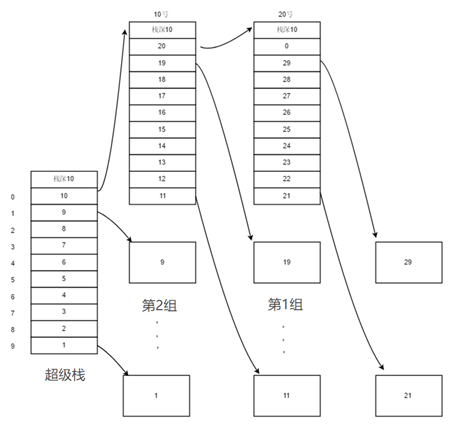代码
数据结构定义
#include<iostream>
#include<queue>
using namespace std;

#define N 10 //最大栈深
#define max 30//最大盘块数

//普通栈定义
struct st
{
int stackDeepth=0; //栈深
int stack[N]; //栈头数组 根据栈深获取对应的盘块
st* next=NULL; //如果该栈满了 则需要新增一个新栈
};

st s_stack; //超级栈   超级栈的栈深s_stack.stackDeepth==s_stackDeepth

//初始化栈
void InitStack(st* Stack){
Stack->next=NULL;
for(int i=0;i<N;i++)
Stack->stack[i]=0;
Stack->stackDeepth=0;
}
//清空化栈
void CleanStack(st* Stack){
Stack->next=NULL;
for(int i=0;i<N;i++)
Stack->stack[i]=0;
Stack->stackDeepth=0;
}
//显示栈表
void showStack(st* stack)
{
int n=0;
cout<<"*******第0号栈是超级栈*******"<<endl;
while(stack!=NULL)
{
//第0号栈是超级栈
cout<<"第"<<n<<"个栈的盘块号,栈深是"<< stack->stackDeepth<<endl;
for(int i=0;i<N;i++)
cout<<"盘块"<<stack->stack[i]<<" ";
cout<<endl;
if(stack->next!=NULL)
stack=stack->next;
else
stack=NULL;
n++;
}
}
// 创建成组链接
//创建成组链接
void makeList()
{
int i,a=9;
int n;
st* tempStack=new st();//定义一个临时的栈 为后续的增加栈准备
st* Captain=new st(); //定义一个临时指针
for(i=1,n=9;i<max+1;i++)
{
//先填满超级栈，再填普通的栈
if(i<N&&n>-1)
{
s_stack.stack[n]=i; //填入盘块号
s_stack.stackDeepth++; //栈深+1
n--;
}
else if(i==N) //i==最大栈深的时候 不用最大号盘来存储 而是作为组长块
{
s_stack.next=tempStack; //指向下一个栈 下一个栈的名字就是stack
s_stack.stackDeepth++; //栈深+1
s_stack.stack=i; //栈底的元素是组长，需要置数
}
else
{
//这里存放的是普通栈的内容
tempStack->stack[a]=i;
tempStack->stackDeepth++;
a--;
if(i%N==0)
{
a=9; //重置一下原来临时栈的栈深盘号
if(i==N) //10特殊 是和超级栈相连的
{
s_stack.next=tempStack; //这里存储的是 地址为0的组
Captain=tempStack; //临时将新栈的指针存放在Cap中 这个栈是超级栈后面的第一组
if(i!=max)
Captain->stack=i; //栈底的元素是组长，需要置数
else
Captain->stack=0; //最后一块栈如果满了，栈底盘块号是0 用来警醒内存满了
tempStack=(st*)malloc(sizeof(st)); //分配一个新地址 新栈地址为1（假设）
InitStack(tempStack);
}
else
{
Captain->next=tempStack; //这里存储的是 地址为1的组
Captain=tempStack; //更新Captain指向地址为1的组，这些都是超级栈后面的二、三、四、、、组
if(i!=max)
Captain->stack=i; //栈底的元素是组长，需要置数
else
Captain->stack=0; //最后一块栈如果满了，栈底盘块号是0 用来警醒内存满了
tempStack=(st*)malloc(sizeof(st)); //分配一个新地址 新栈地址为2（假设） 后面以此类推
InitStack(tempStack);
}
}
else //栈不满的情况下
{
Captain->next=tempStack; //这里存储的是 地址为1的组
}
}
}
cout<<"-------开始创建空闲块成组链接------"<<endl;
showStack(&s_stack);
cout<<"-------创建完成------"<<endl;
}
// 分配磁盘
queue<int> q;   //创建一个int类型的队列q
//分配磁盘
st* Devide(st* stack,int n) //根据数量，减去相应的栈深，以栈深为引，分配相应的磁盘
{
int nm=0;
st* temp=stack;
//判断盘块数是否合理
if(n<=0)
{
cout<<"*******************申请的盘块数不合理******************* "<<endl;
cout<<endl;
return stack;
}
//判断是否足够盘块
while(temp!=NULL)
{
nm+=temp->stackDeepth;
temp=temp->next;
}
if(n>nm)
{
cout<<"*******************申请的盘块大于剩余盘块,当前剩余盘数为 "<<nm<<" *******************"<<endl;
cout<<endl;
return stack;
}
int j=stack->stackDeepth;
int tmp;
cout<<"-------查看已经分配的磁盘号："<<endl;
//从栈顶开始将分配出去的磁盘号入队
for(int i=0;i<n;i++)
{
tmp=j-(i%N)-1;
cout<<stack->stack[tmp]<<" ";
//队列操作 进队
q.push(stack->stack[tmp]);
//栈操作 深度减一 清空
stack->stackDeepth--;
stack->stack[tmp]=0;
//tmp==0说明该栈已经分配完了
if(tmp==0)
{
n=n-i-1;
i=-1;
stack=stack->next;//指向下一个栈
j=stack->stackDeepth; //获取该栈的栈深
}
}
if(!q.empty()) //判断队列是否空
{
cout<<endl;
cout<<"数列非空"<<endl;
cout<<"数列q有"<<q.size()<<"个元素"<<endl;
}

return stack;
}

// 释放磁盘
void Release(st* stack,int n)//根据数量，增加相应的栈深，以栈深为引，将相应的磁盘号加入栈中
{
//先判断此时要释放的盘块是否与已分配的盘块相符
if(n>q.size())
{
cout<<"*******************释放的盘块过多，不合理.**********************"<<endl;
cout<<endl;
return;
}
int j=stack->stackDeepth;
int tmp;
int m1=n-1;//操作数组的变量
cout<<"-------查看已经释放的磁盘号："<<endl;
int t[n];//存储从队里面出来的元素
for(int m=0;m<n;m++)
{
t[m]=q.front();// 首元素
//队列操作 出队 删除首元素
q.pop();
}
//从栈顶开始将分配出去的磁盘号入队
for(int i=0;i<n,m1>=0;i++)
{
tmp=j+(i%N);
//注意：要大的磁盘号先进栈，小的盘号后进栈
//栈操作 进栈 深度加一
//cout<<"------m1------"<<m1<<endl;
stack->stack[tmp]=t[m1];
stack->stackDeepth++;
m1--;

cout<<stack->stack[tmp]<<" ";
//tmp==N-1说明该栈已满
if(tmp==N-1)
{
n=n-i;//去掉刚刚已经进栈的
i=-1;//循环有个i++ 所以本来要令i=0 还要减一
st* tempStack=new st();//分配一个新地址的栈
InitStack(tempStack);
//将当前栈的内容存放到新建的临时栈中
tempStack->next=stack->next;
for(int m=0;m<N;m++)
{
tempStack->stack[m]=stack->stack[m];
}
tempStack->stackDeepth=stack->stackDeepth;

CleanStack(stack);//清空原来的栈（一般操作的都是超级栈）
stack->next=tempStack; //指向新的栈
j=stack->stackDeepth; //获取栈的栈深
}
}
if(!q.empty()) //判断队列是否空
{
cout<<endl;
cout<<"数列非空"<<endl;
cout<<"数列q有"<<q.size()<<"个元素"<<endl;
}
}
// 维护过程
//维护链表(栈内凑连续块) bubble
void SafeStack(st* stack)
{
int temp;
while (stack!= NULL)
{
for (int i = 0; i < N; i++)
{
for (int j = 0; j < N - i; j++)
{
if (stack->stack[j] < stack->stack[j + 1])
{
if(stack->stack==0)
continue;
temp = stack->stack[j];
stack->stack[j] = stack->stack[j + 1];
stack->stack[j + 1] = temp;
}
}
}
stack = stack->next;
}
}

// 主函数
int main()
{
st* b;
//创建成组链表
makeList();
//A分配0个或-1个盘块，没有超出栈深的分配
cout<<endl;
b=Devide(&s_stack,0);
b=Devide(b,-1);
showStack(b);
//A分配5个盘块，没有超出栈深的分配
cout<<endl;
b=Devide(b,5);
showStack(b);
//A分配7个盘块，超出了栈深的分配
cout<<endl;
b=Devide(b,7);
showStack(b);
//A释放13个盘块,超出了已分配的盘块数量，没有那么多盘块可以释放，会失败
cout<<endl;
Release(b,13);
showStack(b);
//A释放11个盘块，正常释放
cout<<endl;
Release(b,11);
showStack(b);
//B分配7个盘块，在A已经分配的基础上，继续分配
cout<<endl;
b=Devide(b,7);
showStack(b);
//B释放两个盘块
cout<<endl;
Release(b,2);
showStack(b);
//A释放两个盘块
cout<<endl;
Release(b,2);
showStack(b);
//维护盘块
cout<<endl;
cout<<"--------维护盘块----------"<<endl;
SafeStack(b);
showStack(b);
//分配的空间超出剩余的空间
//B分配30个空间
cout<<endl;
b=Devide(b,30);
showStack(b);

return 0;
}

结果与分析
实验结果是有针对性的，对于功能各种极限情况进行测试。
运行主函数，根据得到的结果来分析：
1. 创建成组链表
makeList();
运行结果：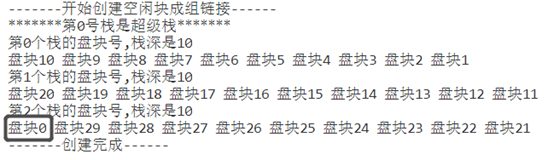分析：
创建的第0个栈是超级栈，分配和释放的操作都首先在超级栈实现。盘块10到盘块1，是盘块号，从左往右即从stack栈底到stack栈顶。
创建过程是从超级栈的stack开始，当超级栈满了，即超过了数组最大的范围N（本次实验的最大栈深是10），则创建新的栈继续存放，并把当前栈的指针next指向新的栈。
当这个成组链接的最大空间是max==30，则第30个盘块号为0，即上图黑框内容。并不表示物理块号，而是分配警戒位，作为空闲盘块连的结束标志。
2. 分配
//申请的盘块数不合理
    //A分配0个或-1个盘块，没有超出栈深的分配

cout<<endl;

b=Devide(&s_stack,0);

b=Devide(b,-1);

showStack(b);

运行结果：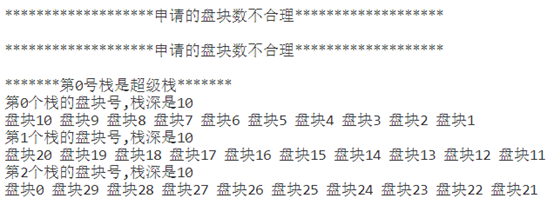分析：
当申请的盘块数是0或者小于0，则为申请不合理。栈链接无变化。
//申请的盘块数不大于超级栈的最大栈深N
    //A分配5个盘块，没有超出栈深的分配

cout<<endl;

b=Devide(b,5);

showStack(b);

运行结果：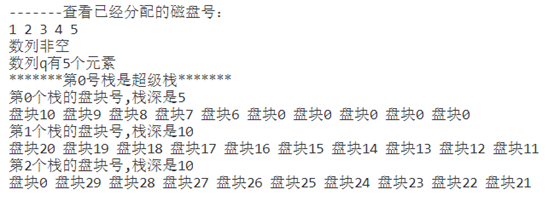分析：
从栈顶开始取出盘块，放进队列中，队列先进去的是1，接着是2，3，4，5.取出之后，原来盘块号置为0，这个地方有待优化，不能和结束标志相同。 超级栈的栈深变为5，数列q中有5个元素。
//申请的盘块数大于超级栈的最大栈深N
//A分配7个盘块，超出了栈深的分配

cout<<endl;

b=Devide(b,7);

showStack(b);

运行结果：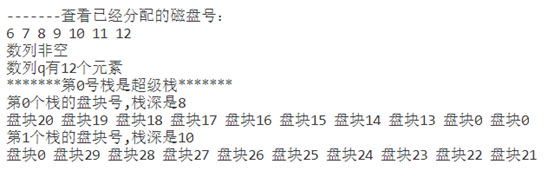分析：
根据上一次分配了5个盘块的结果，超级栈的栈深变为5，小于现在所需要的7个盘块，则需要从下一个栈中补上所需要的盘块数。
可以看到原来的超级栈中5个被分配出去，已经空了，则超级栈中存入下一个栈的内容，下一个栈空间释放。继续分配2个盘块11号和12号。
最后栈链接如上图所示，超级栈的栈深是8，数列q有12个元素，本次分配了6，7，8，9，10，11，12号。
//分配的空间超出剩余的空间
    //B分配30个空间

cout<<endl;

b=Devide(b,30);

showStack(b);

运行结果：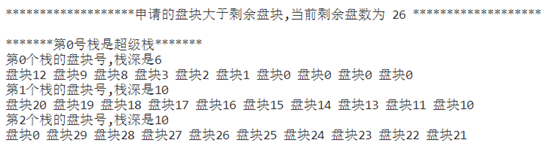分析：
当申请所需的盘块数大于栈链接所剩余的盘块数时，则会提示错误。
实现方法如图所示：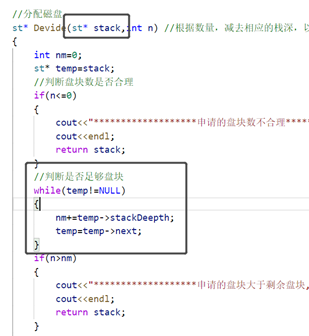3. 释放
//释放的盘块数大于已分配的数量
//A释放13个盘块,超出了已分配的盘块数量，没有那么多盘块可以释放，会失败

cout<<endl;

Release(b,13);

showStack(b);

运行结果：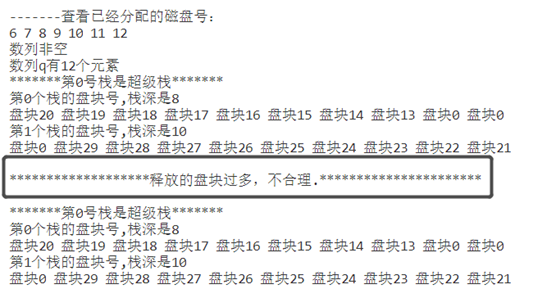分析：
上图黑色框的是代码运行结果。A进程只是申请了12个盘块，没办法释放13个盘块。所以栈链接没有变化，和上一次结果相同。
实现代码：判断释放盘块数n是否大于数列q里的元素数量。
//释放的盘块数合理，超出了超级栈的剩余存放盘块空间数量
//A释放11个盘块，正常释放

cout<<endl;

Release(b,11);

showStack(b);

运行结果：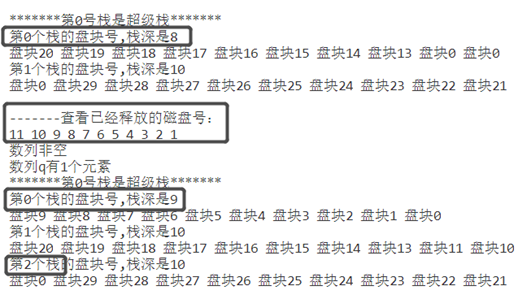分析：
上图中，第一个栈链接只有两个栈，在A进程释放了11个盘块之后，栈链接多了一个栈，是因为所释放的盘块数和超级栈中原来剩下的盘块数之和大于超级栈的最大栈深N.出现这种情况的时候，会创建多一个栈空间，将当前超级栈的内容放进新栈之中。
之后新来的盘块则是新栈的组长，这个组长盘块会存放在超级栈之中，超级栈的指针next会指向新建的栈，新栈的next指针内容会继承超级栈原来的指针内容。接着继续往超级栈中存放剩余的释放出来的盘块。
实验结果可以看出，原来超级栈的栈深是8，存入2个盘块之后，栈满，则新建一个栈（组长号是超级栈的srack），新栈的栈深是10，超级栈的栈深为0.接着超级栈继续存放剩下9个盘块，超级栈的栈深变为9，数列q的元素剩余1个（这里仅有A进程的操作，没有进行B进程的操作，所以数列由12-11==1,变为1个元素）。
// 释放的内容小于当前超级栈剩余的盘块空间
//B释放两个盘块

cout<<endl;

Release(b,2);

showStack(b);

//A释放两个盘块

cout<<endl;

Release(b,2);

showStack(b);

运行结果：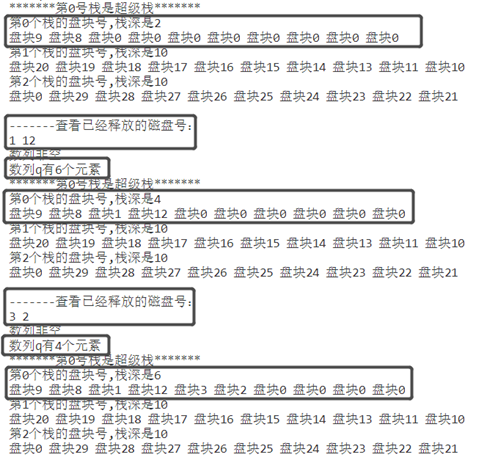分析：
在当前超级栈的空余空间大于释放盘块数时，直接将数列列头的元素取出，栈中存在盘块号大的在盘块号小的上面的问题，所以需要维护。
另外，在申请的盘块数合理的前提下，本次实验不存在释放的盘块数大于栈链接最大盘块空间的问题。
4. 维护盘块
//将磁盘乱序的盘块，凑成连续块
 SafeStack(b);
运行结果：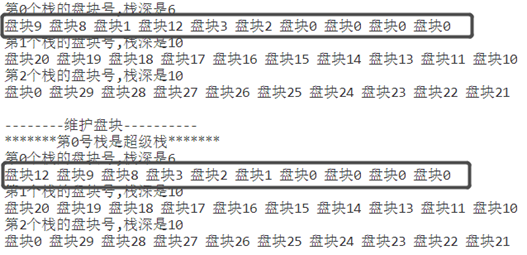分析：
盘块在释放和分配的过程中，必定会尝试不连续的盘块号，例如上图中第一个框框所示。我是用排序算法将盘块号按栈顶比栈底小的顺序排列。
目前实现的算法的缺陷是，只能在本栈内排序，两个栈之间的算法还没能够实现，这可以是未来改进的地方。
另外的补充知识：
C++简单队列结构：link https://blog.csdn.net/hao_ge_666/article/details/96481046
展开全文c++ 操作系统
• 1.设计合适的数据结构模拟磁盘空闲块的情况（课本5.4下的第4点）。 2. 模拟分配空闲块的过程。 3. 模拟回收空闲块的过程。 4. 模拟对所有空闲块进行分析、凑连续块 的维护过程。 总体思路：用二维数组模拟每个盘块 #...
1.设计合适的数据结构模拟磁盘空闲块的情况（课本5.4下的第4点）。 2. 模拟分配空闲块的过程。 3. 模拟回收空闲块的过程。 4. 模拟对所有空闲块进行分析、凑连续块 的维护过程。
总体思路：用二维数组模拟每个盘块
#include<iostream>
#include<vector>
#include<algorithm>
using namespace std;

#define average  6 /*宏定义一组的盘块的块数目*/

int startDisk;//起始盘块号
int numDisk;//初始的总盘块的个数

struct Disk{/*盘块的结构， 即盘号和栈*/
int id;//硬盘号
vector<int>st;//利用向量来模式栈
};

vector<vector<Disk>>allocate;//二维向量模拟盘块成组链接
vector<Disk>used; //已使用的盘块
vector<int>superBlock; //向量模拟超级块

bool cmp(Disk a, Disk b){/*重载向量sort排序函数
按照硬盘号从大到小排序*/
return a.id > b.id;
}

void init(){/*初始化各组以及组长块的栈*/

int start = startDisk;//起始盘号
int end = startDisk + numDisk - 1; //终止盘号
int cycle = 0;//周期，控制一组的盘的个数

vector<Disk>firstGroup; //第一组

Disk fake;//一个“假盘”，即盘号为0的盘
fake.id = 0;//首号块是分配警戒位

firstGroup.push_back(fake);//把“假盘”放在allocate这个位置

while(end >= start && cycle < average - 1){/*构造第一组
注意第一组的盘块最多为
average -1 个盘块， average
是宏定义的每一组的盘块的个数*/
Disk tmp;
tmp.id = end;/*盘号从大到小来填到allocate这个二维数组中*/
end--;
cycle++;
firstGroup.push_back(tmp);
}

allocate.push_back(firstGroup);/*完成第一组的构造*/

while(end >= start){/*将剩余的盘块分组*/
cycle = 0;
vector<Disk>tmpDimension;/*构造这一组*/
for( ; end >= start && cycle < average; ){/*每组最多是average 个盘块*/
Disk tmp;
tmp.id = end;
cycle++;
end--;
tmpDimension.push_back(tmp);
}
allocate.push_back(tmpDimension);/*完成新的一组的构造*/

}

int size = allocate.size();/*二维数组的行数， 即 组 的个数*/

for(int i = 1; i <= size - 1; i++){/*构造从第2组起的每组的组长块的栈*/
allocate[i].st.push_back( allocate[i - 1].size());//填写栈深，即前一组盘块的总数
for(int j = 0; j < allocate[i -1].size(); j++){/*栈填写的内容是前一组盘号*/
allocate[i].st.push_back(allocate[i -1][j].id);/*填写盘号*/
}

}

/*下面是构造超级块*/
int lastGroupSize = allocate[size - 1].size();//最后一组的盘块个数
superBlock.push_back(lastGroupSize);//超级块的栈深就是最后一组的盘块的个数

for(int j = 0; j < lastGroupSize; j++){/*把最后一组的盘块的id写到超级块里面*/
superBlock.push_back(allocate[size - 1][j].id);
}

printf("每组的块数目是 %d 空闲块成组链接初始化完成\n", average);

}

void printEveryGroup(){/*打印每一组的情况*/
int size = allocate.size();/*组的个数*/
if(size <= 0 || (allocate.size() ==1 )){/*allocate.size==1就是
只有allocate这个“假块”
即id为0的块， 就是没有空闲块了！！*/
cout<<"全部盘块已经分配完了， 当前没有剩余盘块"<<endl;
cout<<endl;
return ;
}

for(int i = 0; i < allocate.size(); i++){//每组
cout<<"第 "<< i + 1<<" 组"<<endl;
for(int j = 0; j < allocate[i].size(); j++){//每组的每一块
if(allocate[i][j].id != 0){///这个块不是第一组的结束块
if(j == 0){//每行的首个就是组长块
cout<<"(组长块)"<<allocate[i][j].id<<" ";
}
else
cout<<allocate[i][j].id<<" ";
}
}
cout<<endl;
}

}

void printSuperBlock()/*打印超级块*/
{

int size = superBlock.size();//超级块的长度
//cout<<"栈深："<<superBlock.size() -1 <<endl;
if(size <= 0){
cout<<"有异常，超级块前面init()没有初始化成功"<<endl;
abort(); //中断程序
exit(1);
}
cout<<"栈深"<<superBlock<<endl;
cout<<"栈存储的块号(超级块存储的块号) ";
for(int i = 1; i < size; i++){//下标从1开始才是超级块的存储的块号
cout<<superBlock[i]<<" ";

}
cout<<endl;

}

void assignment(int num){/*分配num个盘块*/

if(num == 0){/*判断参数合法性*/
cout<<"请输入大于0的数字！！"<<endl;
return ;
}

/*计算剩余盘块， 如果不够的话就不要分配出去了*/
int rest = 0; //剩余盘块的个数
for(int i = 0; i < allocate.size(); i++){//计算剩余盘块的个数
for(int j = 0; j < allocate[i].size(); j++){
rest++;
}
}
rest -= 1; /*注意减少1是因为之前统计rest的时候把id 为0 这个假块
算上去了，此时要减去这个 */

if(rest < num ){/*剩余的不够*/
printf("剩余的块是 %d 块， 不够需求，不分配(分配失败)\n", rest);
return ;
}

cout<<"分配的盘块号是: "<<endl;

while(num--){/*一个一个的分配*/
int size = allocate.size();//组的个数
if(superBlock.size() && superBlock >= 2){/*当前空闲块个数超过2
不需要把组长块分配出去*/
int tmp = superBlock.back();/*获取超级块的栈顶(这个是用向量模拟栈)，
即获取需要分配出去的块号*/

if(tmp == 0){/*分配到警告块*/
cout<<"警告， 空闲块已经用光了,无法分配"<<endl;
return ;
}

cout<<tmp<<" ";//输出块号
superBlock.pop_back();//分配出去，弹出栈
used.push_back(allocate[size - 1].back());//放到已使用那里(即标记其已经被使用)
allocate[size - 1].pop_back();//把其在空闲块组中删除，即在最后一组中删除
superBlock--;//更改栈深度
}
else{//要把组长块分配出去
int tmp = superBlock.back();

if(tmp == 0){//无空闲块分配
cout<<"警告， 空闲块都已经用光了,无法分配"<<endl;
return ;
}

superBlock.clear();//清除超级块的内容
superBlock.shrink_to_fit();//回收超级块的内存

//下面的for是开始把组长块的内容复制到超级块中
for(int j = 0; j < allocate[size - 1].st.size(); j++){
superBlock.push_back(allocate[size - 1].st[j]);

}

/*把组长块的栈清空并释放内存*/
allocate[size -1].st.clear();
allocate[size -1].st.shrink_to_fit();

used.push_back(allocate[size -1]);//组长块放到使用表中
allocate.pop_back();//删除该组
allocate.shrink_to_fit();//一定要销毁空间！！

cout<<tmp<<" ";//输出分配的盘号

}
}

cout<<endl;
cout<<"分配完毕"<<endl;

}

bool insertMatrix(const int & num ){/*将释放的空闲块放到组中，即二维矩阵(向量)
allocate模拟的空闲块成组链接中*/

int markPos;
int depth = superBlock; //栈深
if(depth < average ){/*栈未满*/

int i;
for(i = 0; i < used.size(); i++){/*找到要释放的空闲块
在used这个数组的位置
，这是为了将其从used删除，
表示其恢复为空闲了*/
if(used[i].id == num ){
markPos = i;
break;
}
}

if(i == used.size()){
cout<<"输入错误，该块是空闲块或者是不存在的块，请输入已使用的块！！"<<endl;
return false; //返回释放失败
}

superBlock.push_back(num);//更改超级块，把释放的块的盘号放到超级块里面去
superBlock++;//修改栈深

int size = allocate.size();//组的个数

allocate[size -1].push_back(used[markPos]);//放回到最后一组

vector<Disk>:: iterator it = used.begin() + markPos;//指向其在used的位置
it = used.erase(it);//将其从used 数组中删除

}
else{
vector<Disk>dimension; //另起一组

int i;
for(i = 0; i < used.size(); i++){/*找到释放的块其在used 的位置*/
if(used[i].id == num ){
markPos = i;
break;
}
}

if(i == used.size()){
cout<<"输入错误，该块是空闲块，请输入已使用的块！！"<<endl;
return false;
}

//修改栈，首先将栈的内容复制到新的块
for(int j = 0; j < superBlock.size(); j++){
used[markPos].st.push_back(superBlock[j]);

}

dimension.push_back(used[markPos]);/*把这个释放了的块放到新的一组*/
allocate.push_back(dimension);

//重新构造超级块
superBlock.clear();//清空超级块的内容
superBlock.shrink_to_fit();//回收超级块的内存

superBlock.push_back(1);//修改超级块的栈深
superBlock.push_back(used[markPos].id);// 把新的块的id写入到超级块中

vector<Disk>:: iterator it = used.begin() + markPos;//将其从已使用队列删除
it = used.erase(it);

}

return true; //释放的块成功放回到组中
}

void releaseBlock(){/*释放块*/
int num;
int size = used.size();
if(size <= 0){
cout<<"当前没有已经被使用的块(即所有的盘块是空闲的), 无盘块可释放"<<endl;
return ;
}
printf("当前已使用的盘块的总个数是 %d ,已使用的盘的盘号如下\n", size);

for(int i = 0; i < used.size(); i++){/*打印已使用的块的长度*/
cout<<used[i].id<<" ";
}

cout<<endl;

cout<<"请输入回收的盘块的块号，输入 -1 表示停止输入"<<endl;
cin>>num;
while(num != -1 ){
bool check = insertMatrix(num);//回收盘号为num的块
if(check){
cout<<num<<" 号块回收成功"<<endl;
//num--;
}
cin>>num;
}
return ;

}

void reconfigure(){/*重构空闲块成组链接，凑连续块*/

vector<Disk>table;//暂时存储空闲块

for(int i = 0; i < allocate.size(); i++){/*把每一组的块放到table这个数组中*/
for(int j =0; j < allocate[i].size(); j++){
if(allocate[i][j].id != 0)
table.push_back(allocate[i][j]);
}
}

sort(table.begin(), table.end(), cmp);//按照盘号从大到小来排序

while(allocate.size()){/*清空成组链接这个二维数组*/
allocate.pop_back();
allocate.shrink_to_fit();
}

//重新开始分组
int cycle = 0; //控制一组的块数
int length = table.size();//空闲块的个数
int p = 0;//指向空闲块的指针
vector<Disk>firstGroup;//构造第一组

Disk fake; //假块， id为0
fake.id = 0 ;
firstGroup.push_back(fake);/*加入id为0的块，这个只是为了占用allocate这个位置
其实就是空闲盘块链的结束标志*/

while(p < length && cycle < average - 1 ){/*重组第一组第一组最多有 average -1
个，因为第一组没有组长块的*/
firstGroup.push_back(table[p]);
p++;
cycle++;
}

allocate.push_back(firstGroup);//加入二维数组里面去，完成第一组的构造

cycle = 0;//周期清零
while(p < length ){/*构造第2.....n 组*/

vector<Disk>newGroup;//新的一组
while(p < length  && cycle < average ){
newGroup.push_back(table[p]);//丢到新的newGroup这一组里面去
p++;
cycle++;

}
allocate.push_back(newGroup);//丢到二维数组里面，成为空闲成组链接的一组
cycle = 0;
}

//先把组长块的栈内容清空， 以构造全新的栈!!
for(int i = 0; i < allocate.size(); i++){
allocate[i].st.clear();//清除内容
allocate[i].st.shrink_to_fit(); //回收内存
}

for(int i =1 ; i<allocate.size(); i++){/*填写全部组长块的栈深
即为前一组的盘号的个数*/
allocate[i].st.push_back(allocate[i - 1].size());
}

for(int i = 1; i < allocate.size(); i++){/*从第二组开始填写组长块的栈内容
是上面已经完成了栈深的填写*/
for(int j = 0; j < allocate[i -1].size(); j++){
allocate[i].st.push_back(allocate[i -1][j].id);
}

}

superBlock.clear();//清除旧的超级块的内容
superBlock.shrink_to_fit();//销毁旧的超级块的内存
int size = allocate.size();/*组的个数，allocate[size -1][]就是成组链接的最后一组*/
superBlock.push_back(allocate[size -1].size());/*超级块的栈深*/
for(int j = 0; j < allocate[size -1].size(); j++){/*把最后一组的硬盘的块号写到超级块里面去*/
superBlock.push_back(allocate[size -1][j].id);
}

cout<<"重组完成"<<endl;
return ;

}

int main()
{
cout<<"输入空闲盘块的起始盘号 和 空闲盘块的个数 "<<endl;
cin>>startDisk>>numDisk;
cout<<"空闲盘块的块号的范围是： "<<startDisk<<"---"<<startDisk + numDisk - 1<<endl;

init();//初始化，构造成组链接。

int choice; //选择

while(true){
printf("【1】 输入 数字 1 是分配盘块\n");
printf("【2】 输入 数字 2 是回收盘块\n");
printf("【3】 输入 数字 3 是重组空闲块盘块\n");
printf("【4】 输入 数字 4 打印每个组的分配情况\n");
printf("【5】 输入 数字 5 打印超级块的情况\n");

cin>>choice;
if(choice == 1){
int num;
cout<<"请输入需要分配的块的个数"<<endl;
cin>>num;
assignment(num);//分配num个块
continue ;
}

if(choice == 2){
releaseBlock();//回收块
continue;

}
if(choice == 3){
cout<<"重组空闲块"<<endl;
reconfigure();//重组空闲块，使得每一组的块号更加连续
continue ;

}
if(choice == 4){
printf("---------打印各个组的情况-------------\n");
printEveryGroup();
continue ;

}

if(choice == 5){/*打印超级块的情况*/
printSuperBlock();
continue ;
}

}

system("pause");

}

展开全文• 一、成组链接法是UNIX/Linux等大型文件系统采用的文件空间管理方法。在UNIX/Linux系统中，将空闲块分成若干组，每100个空闲块为一组，每组的第一个空闲块登记了下一组空闲块的物理盘块号和空闲块总数。如果一组的第...
• 成组链接法 0.思维导图 1.存储空间的划分与初始化 2.空闲表法 如何分配？ 如何回收？ 3.空闲链表法 空闲盘块链 空闲盘区链 4.位示图法 如何分配与回收？ 5.成组链接法 超级的作用 如何分配？ 需要...


文章目录
0.思维导图1.存储空间的划分与初始化2.空闲表法3.空闲链表法空闲盘块链空闲盘区链
4.位示图法5.成组链接法

0.思维导图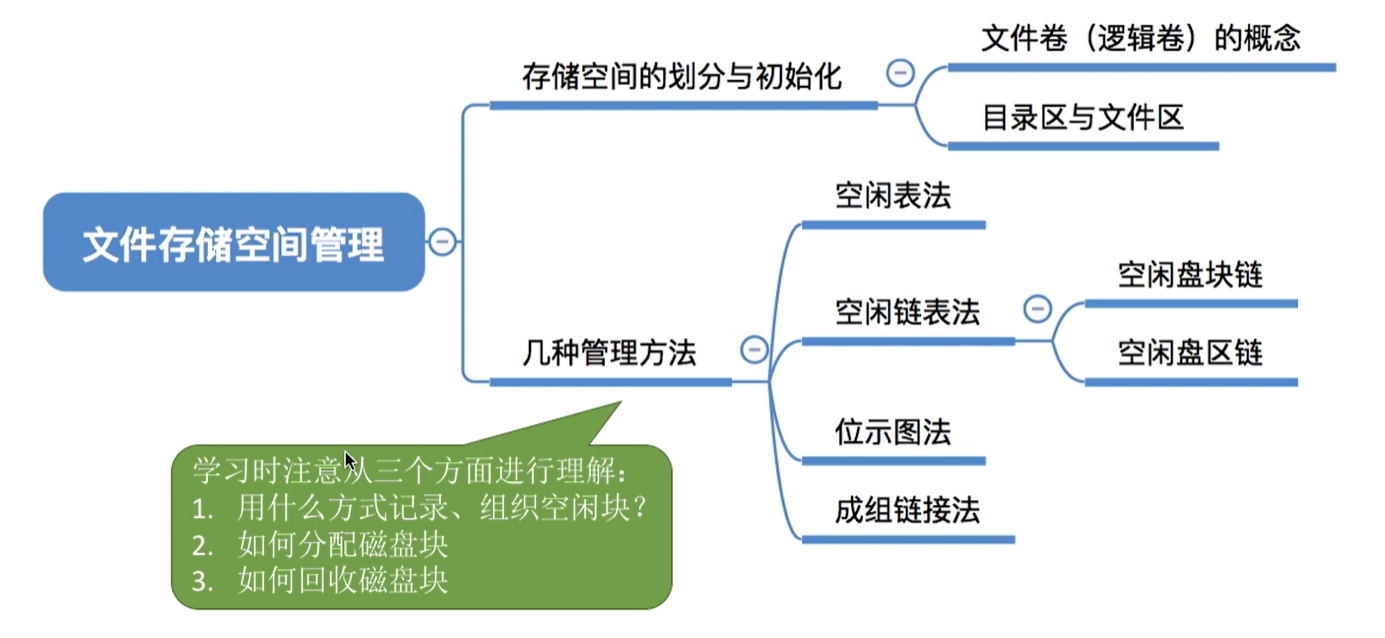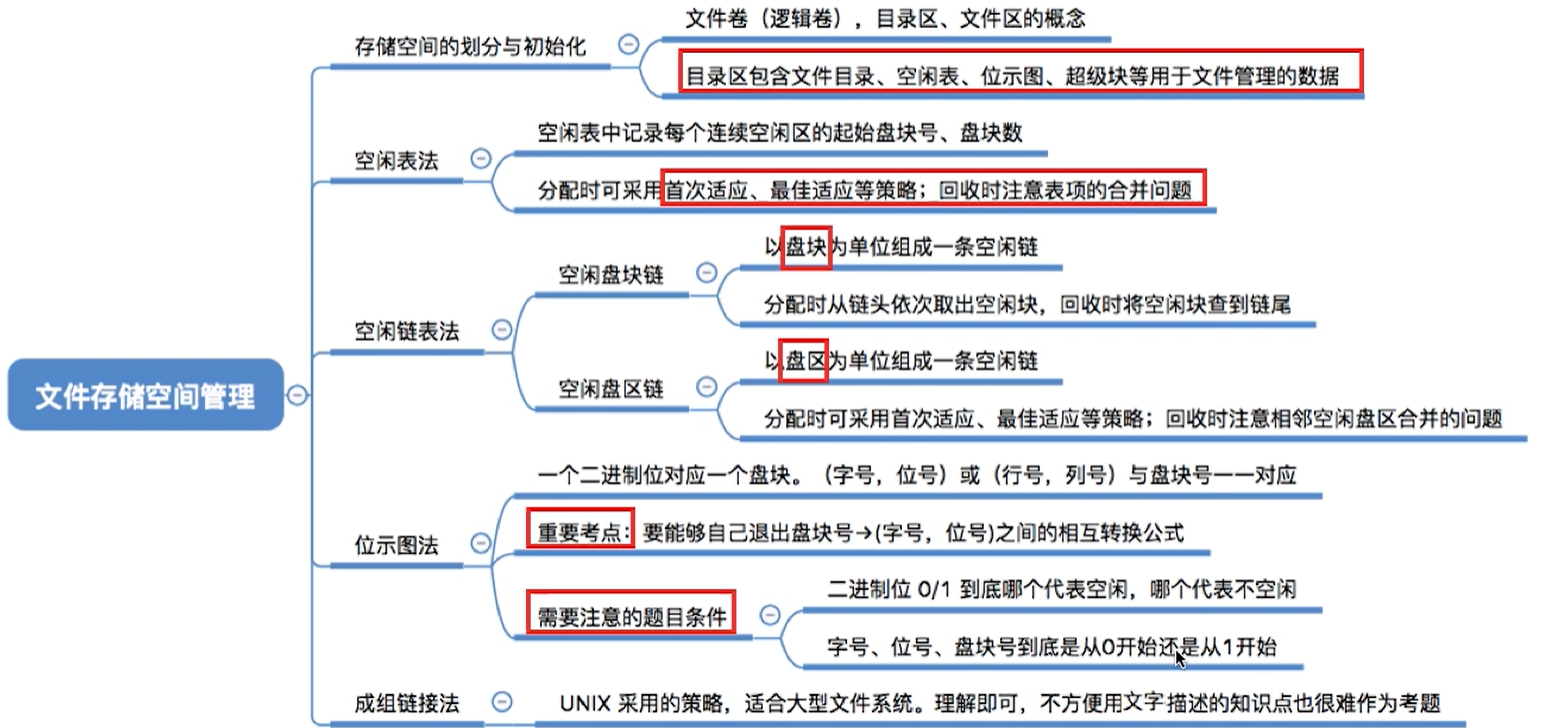1.存储空间的划分与初始化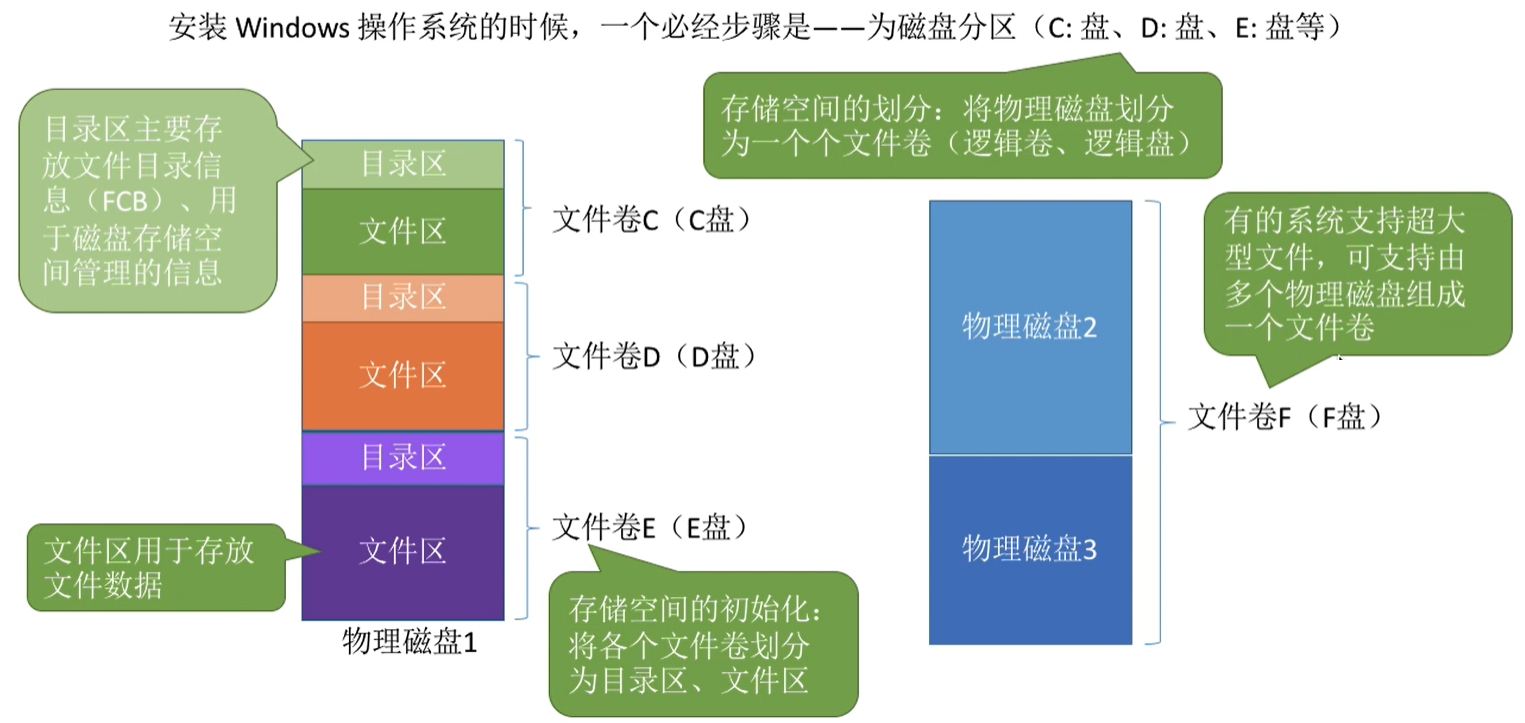2.空闲表法
如何分配？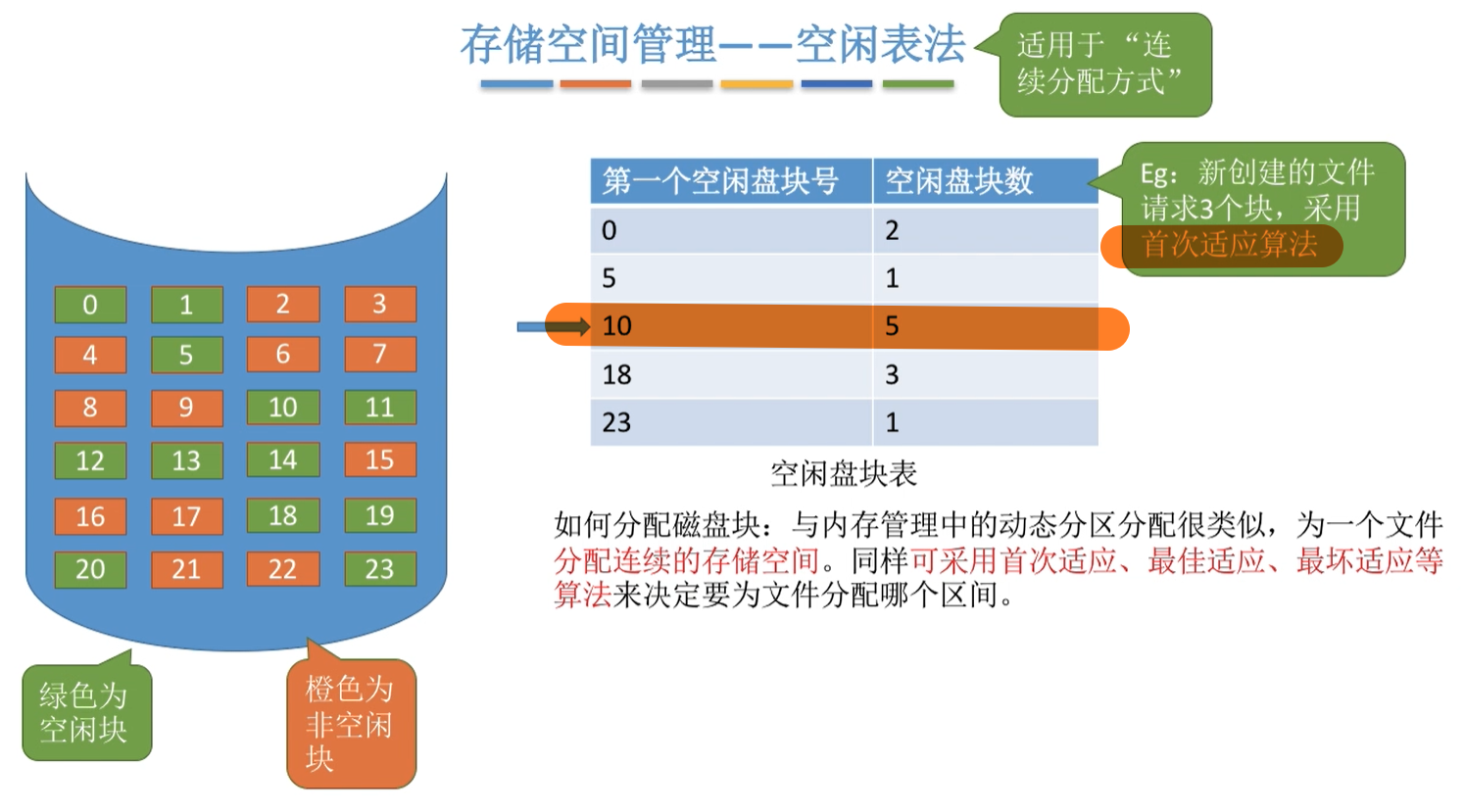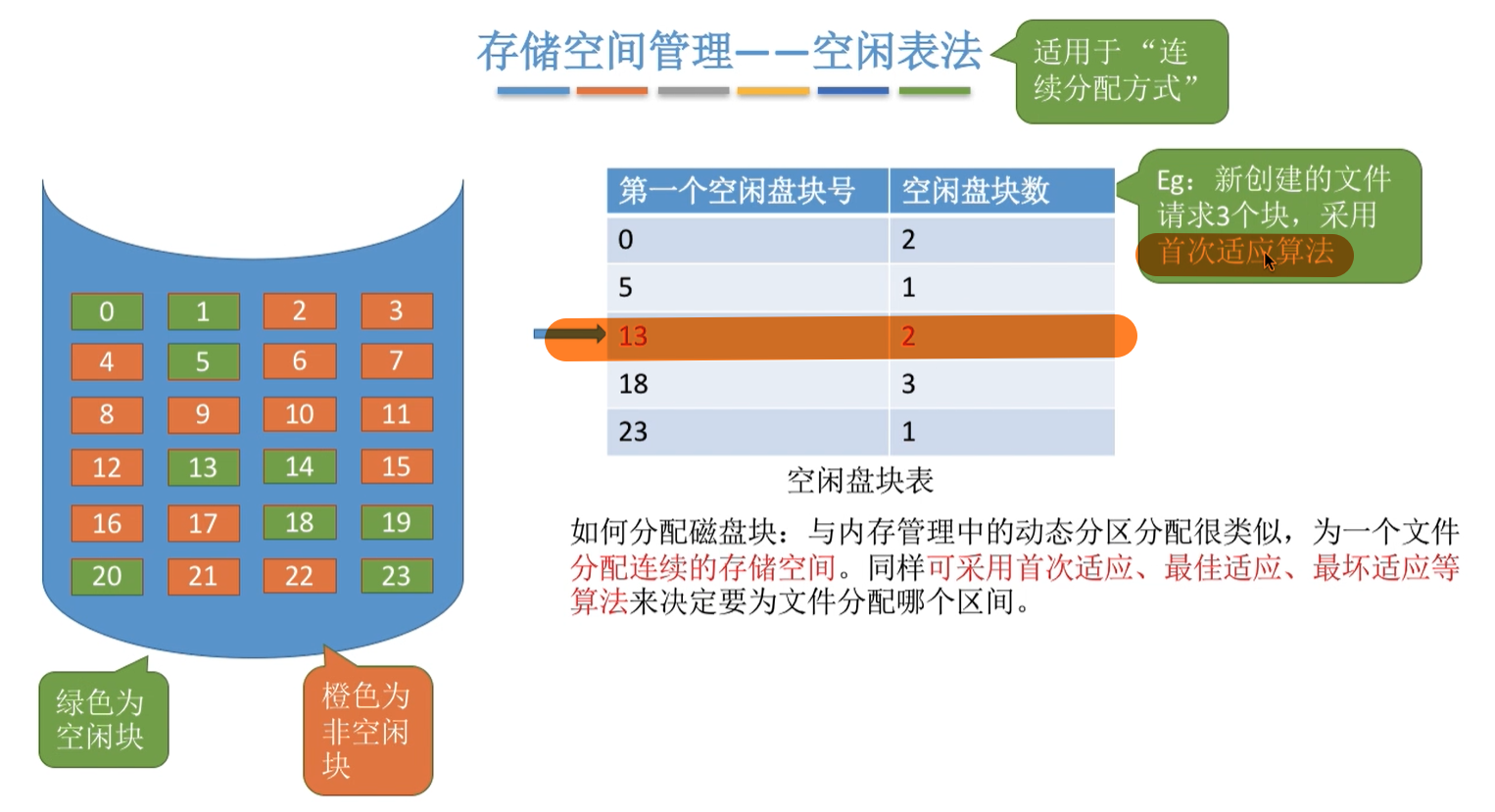如何回收？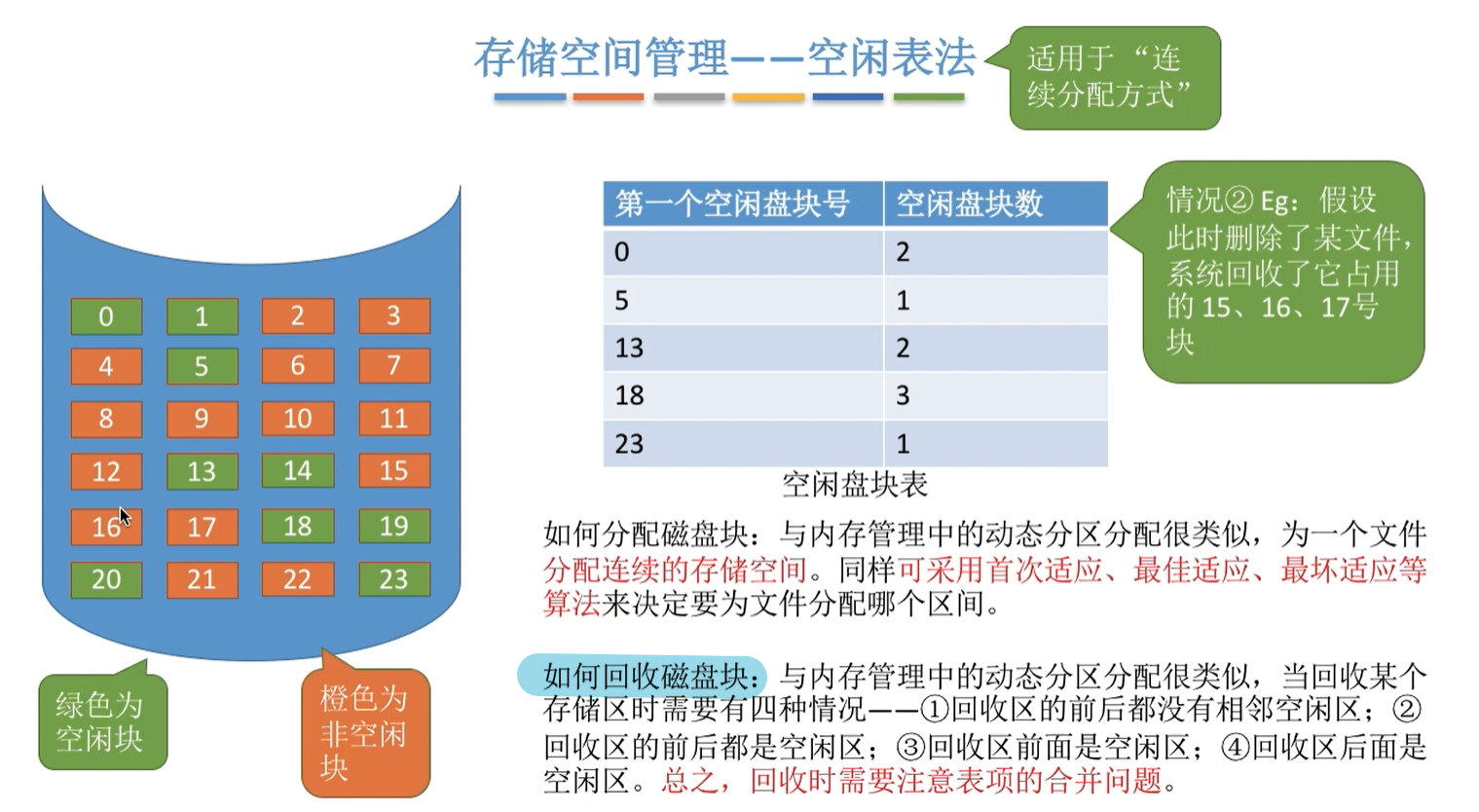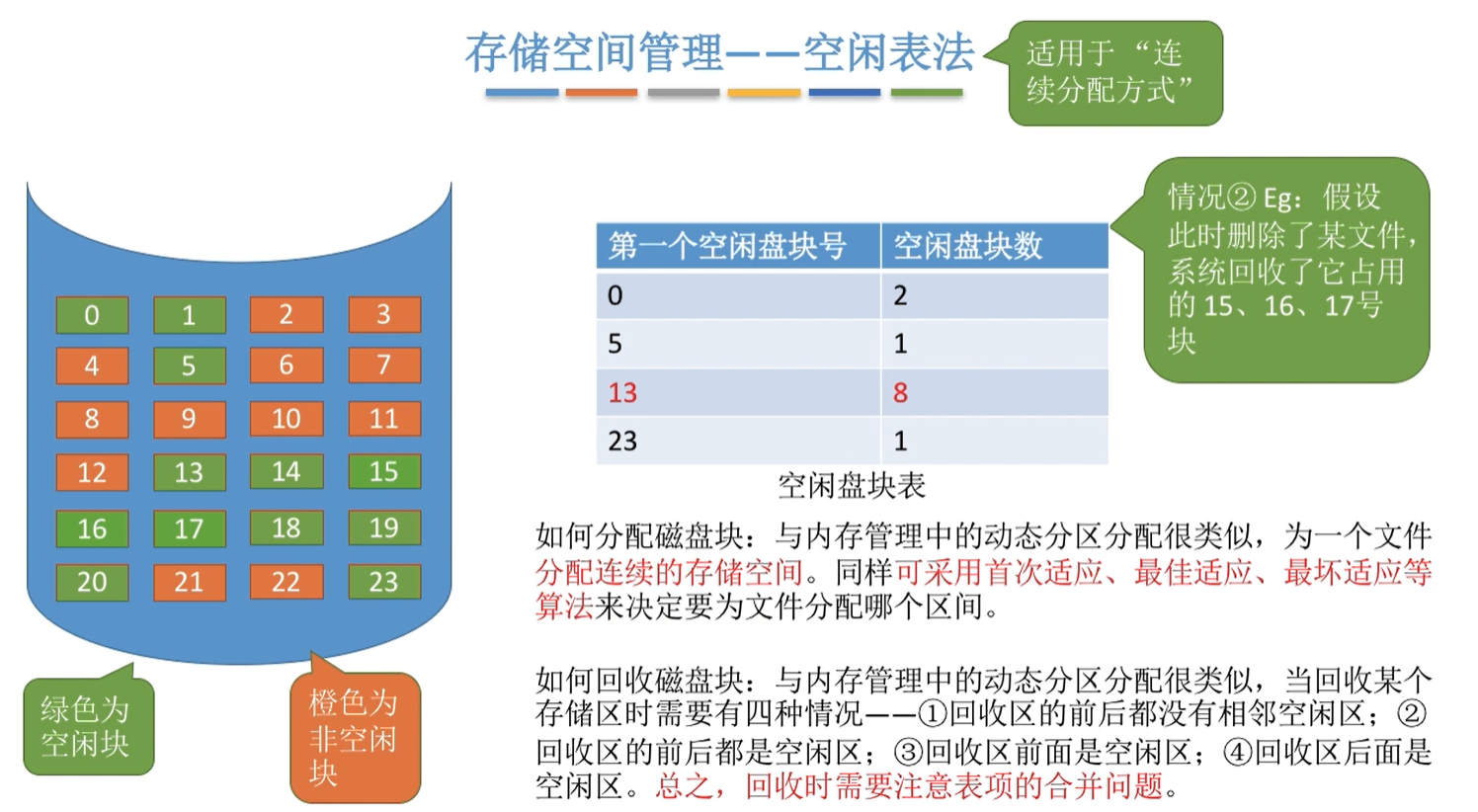3.空闲链表法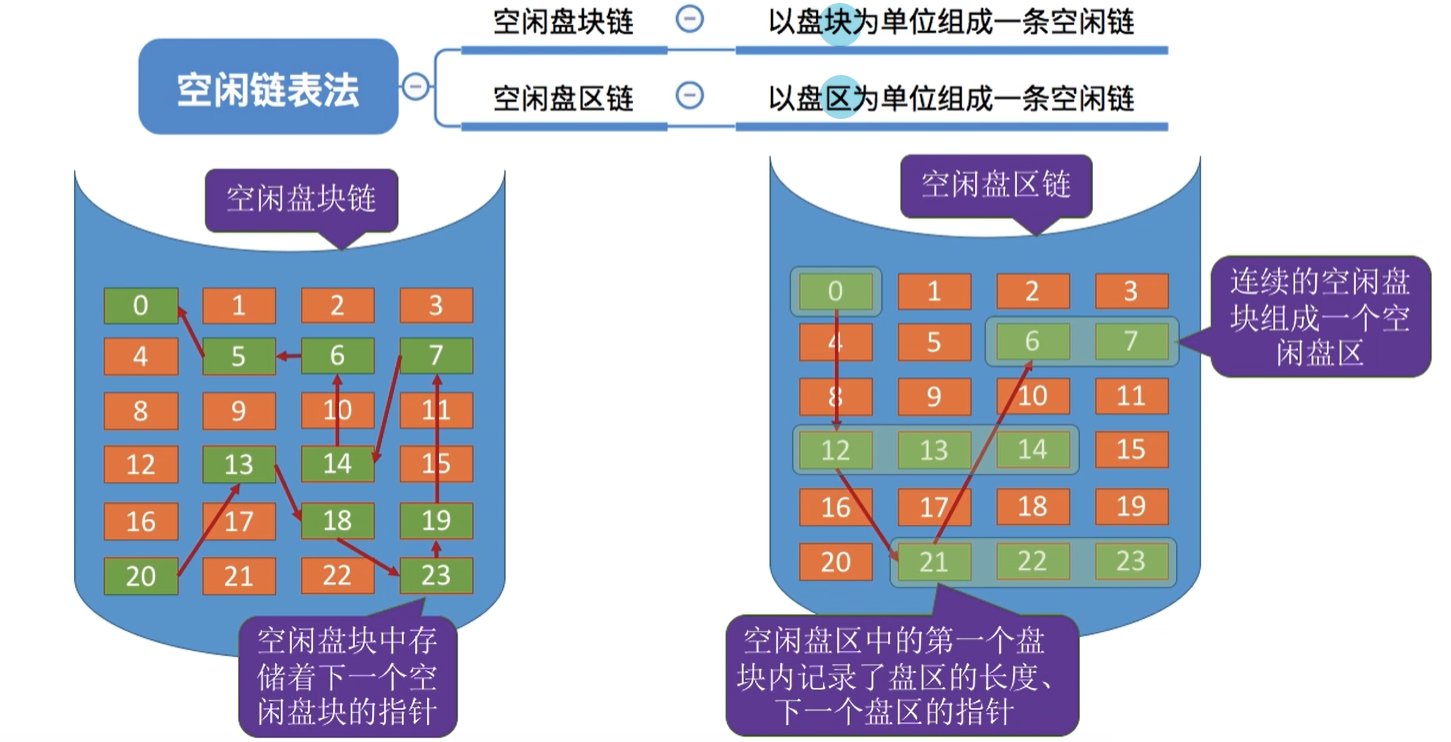空闲盘块链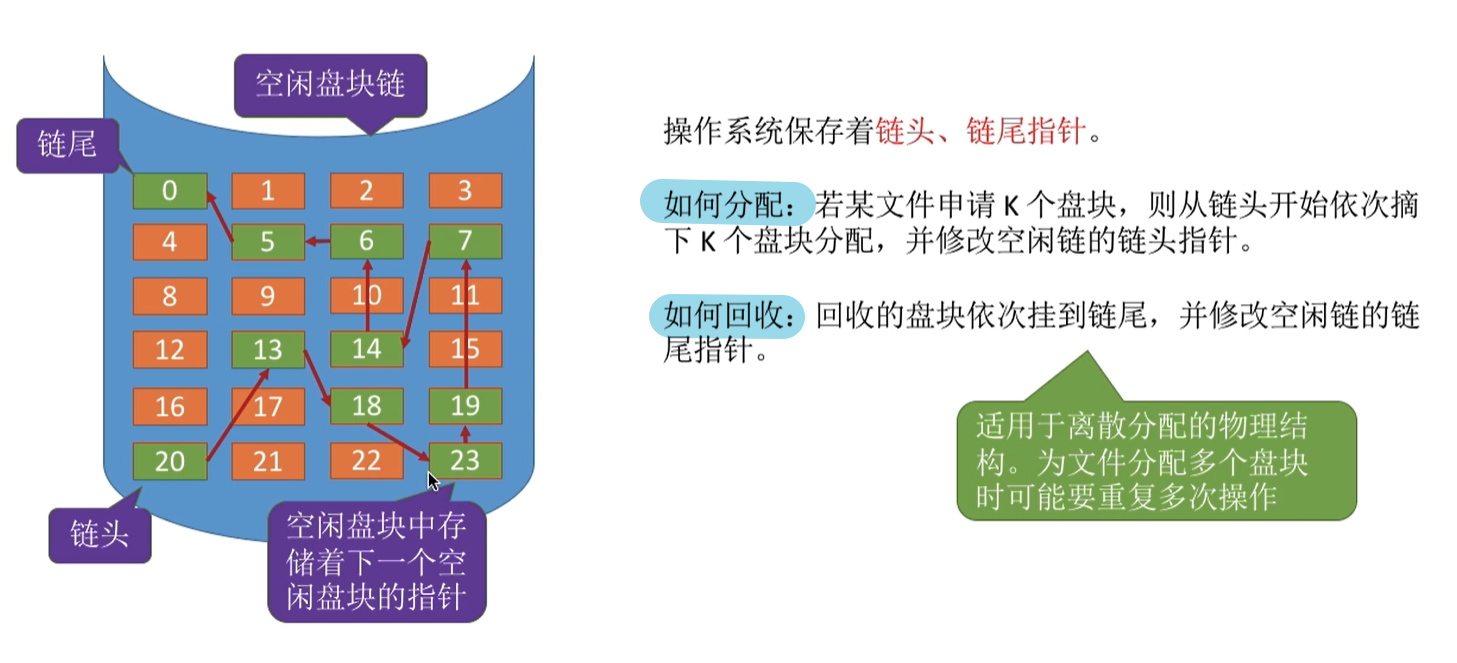空闲盘区链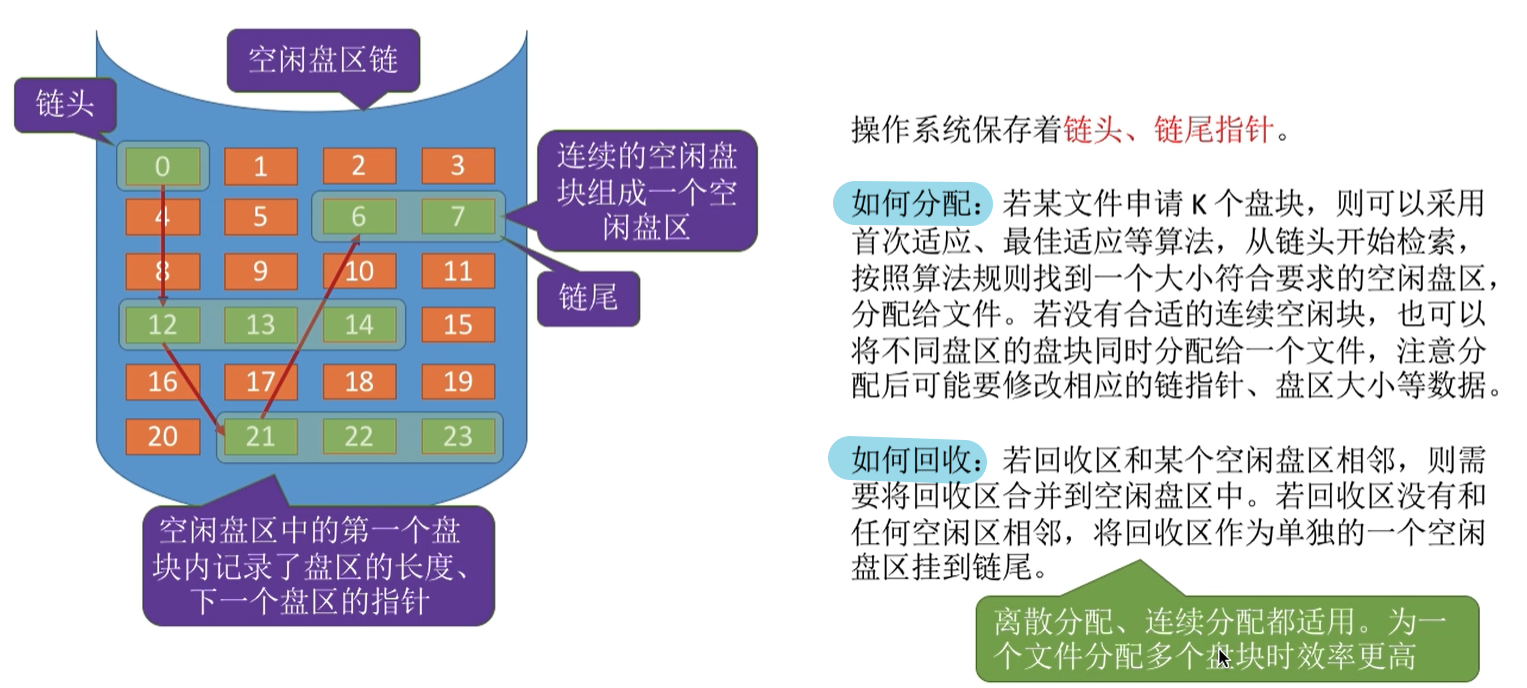4.位示图法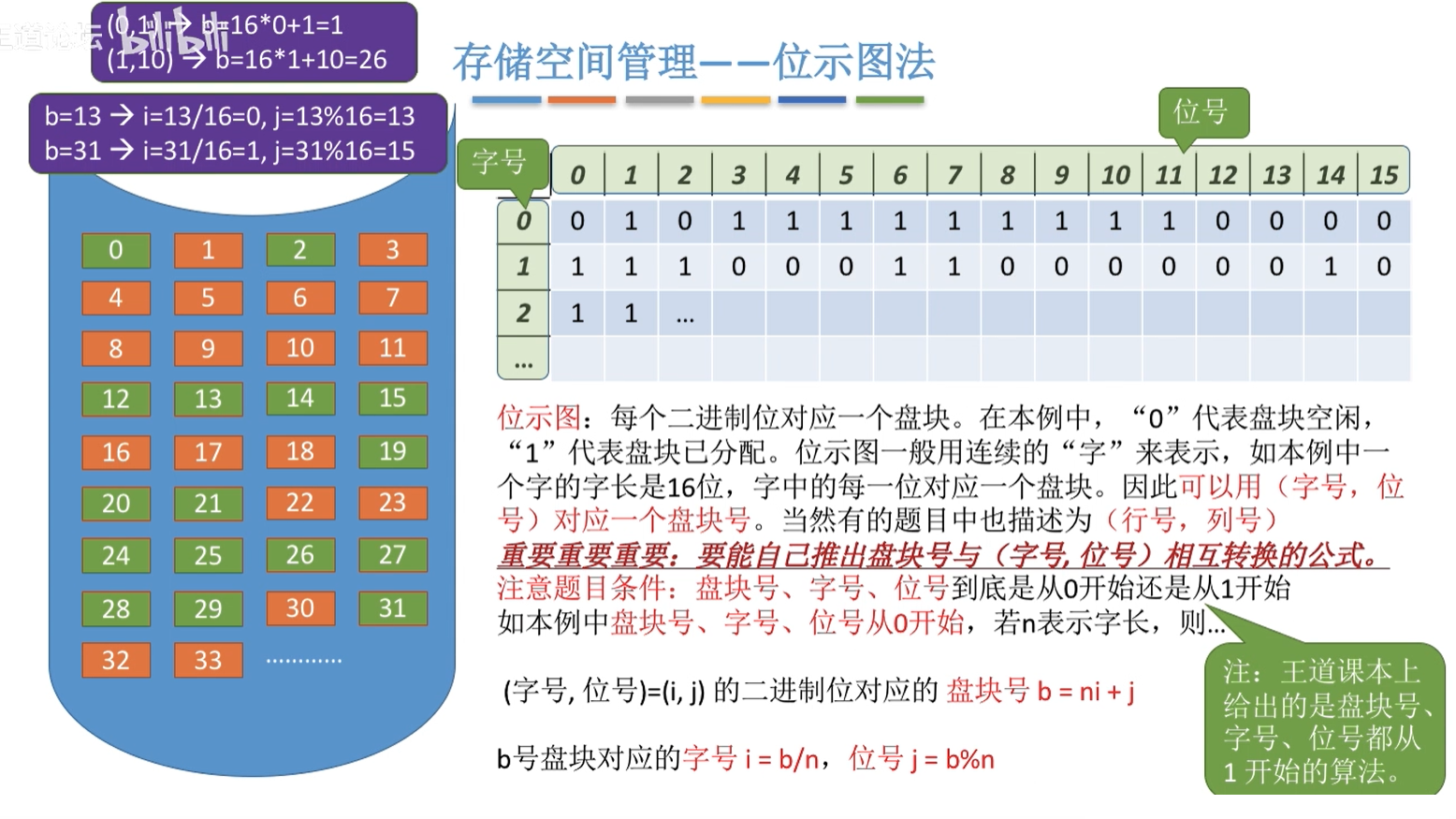如何分配与回收？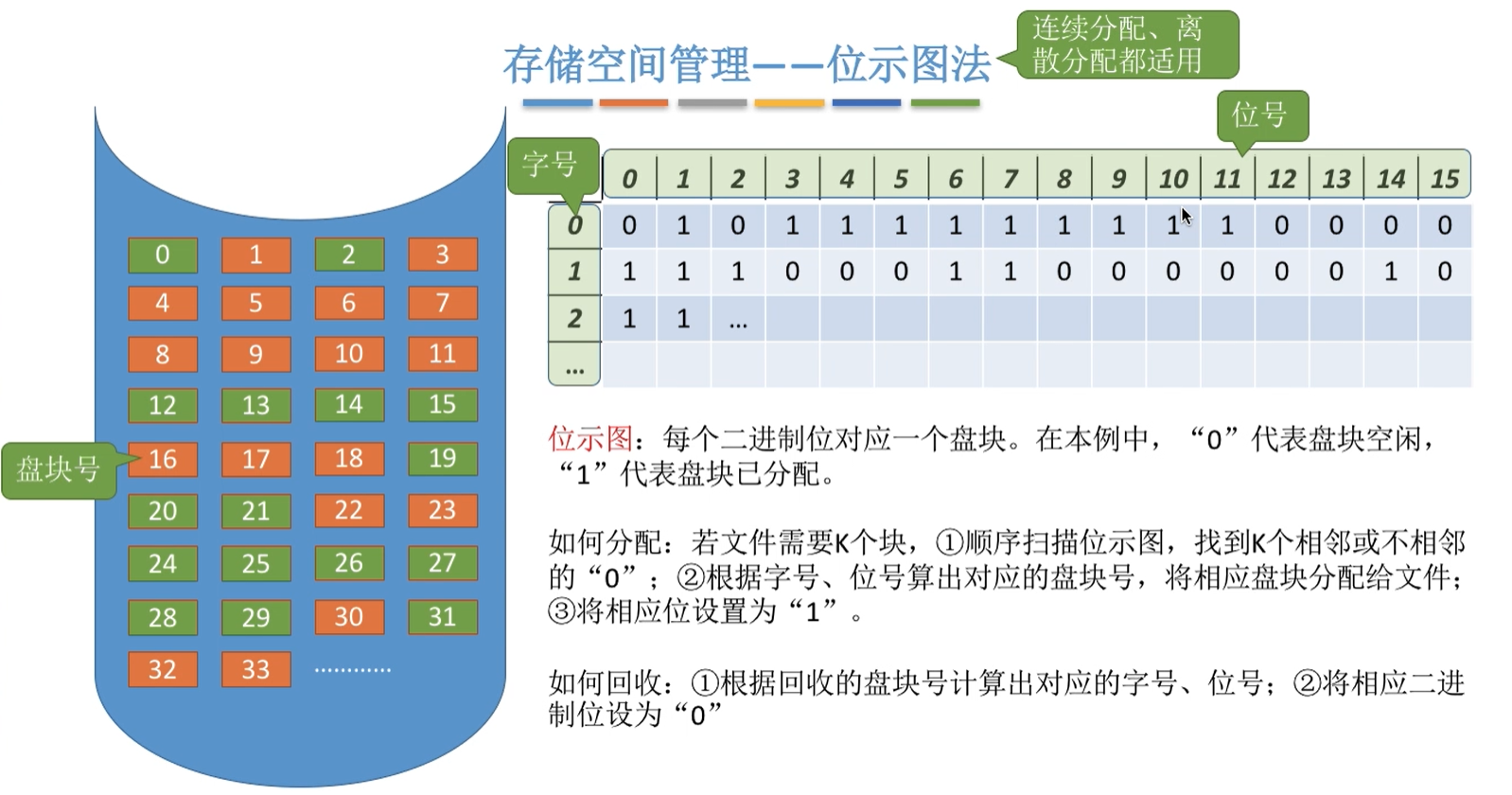5.成组链接法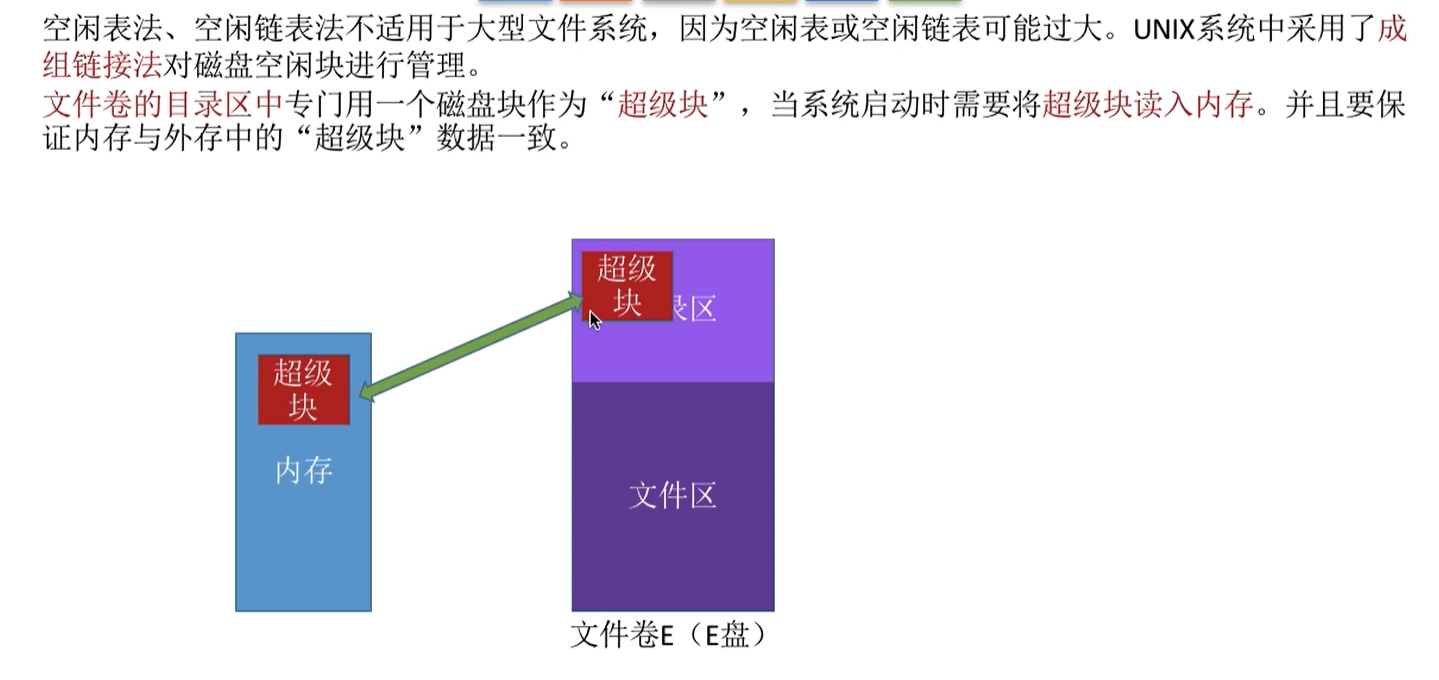超级块的作用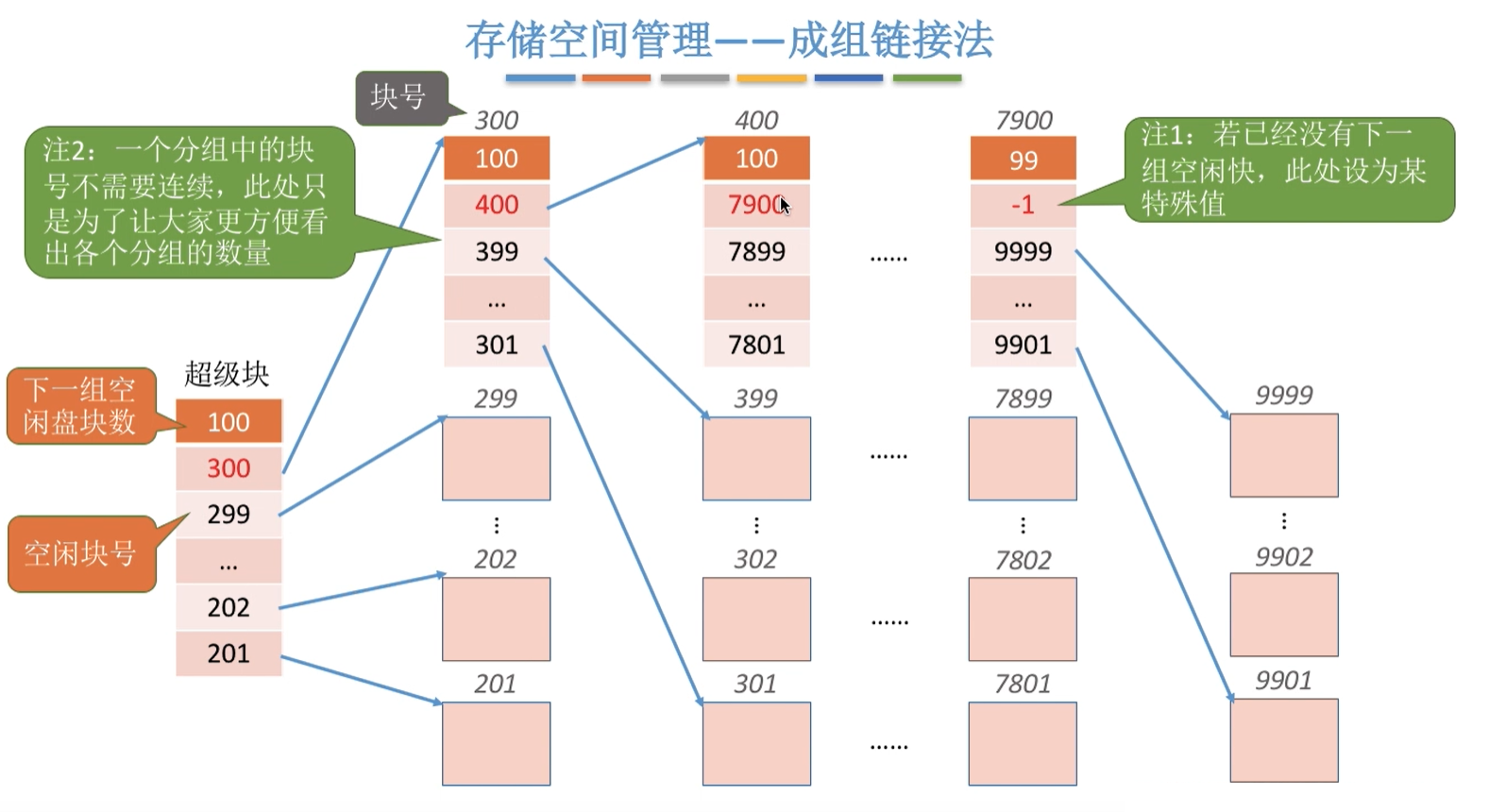如何分配？ 需要1个空闲磁盘块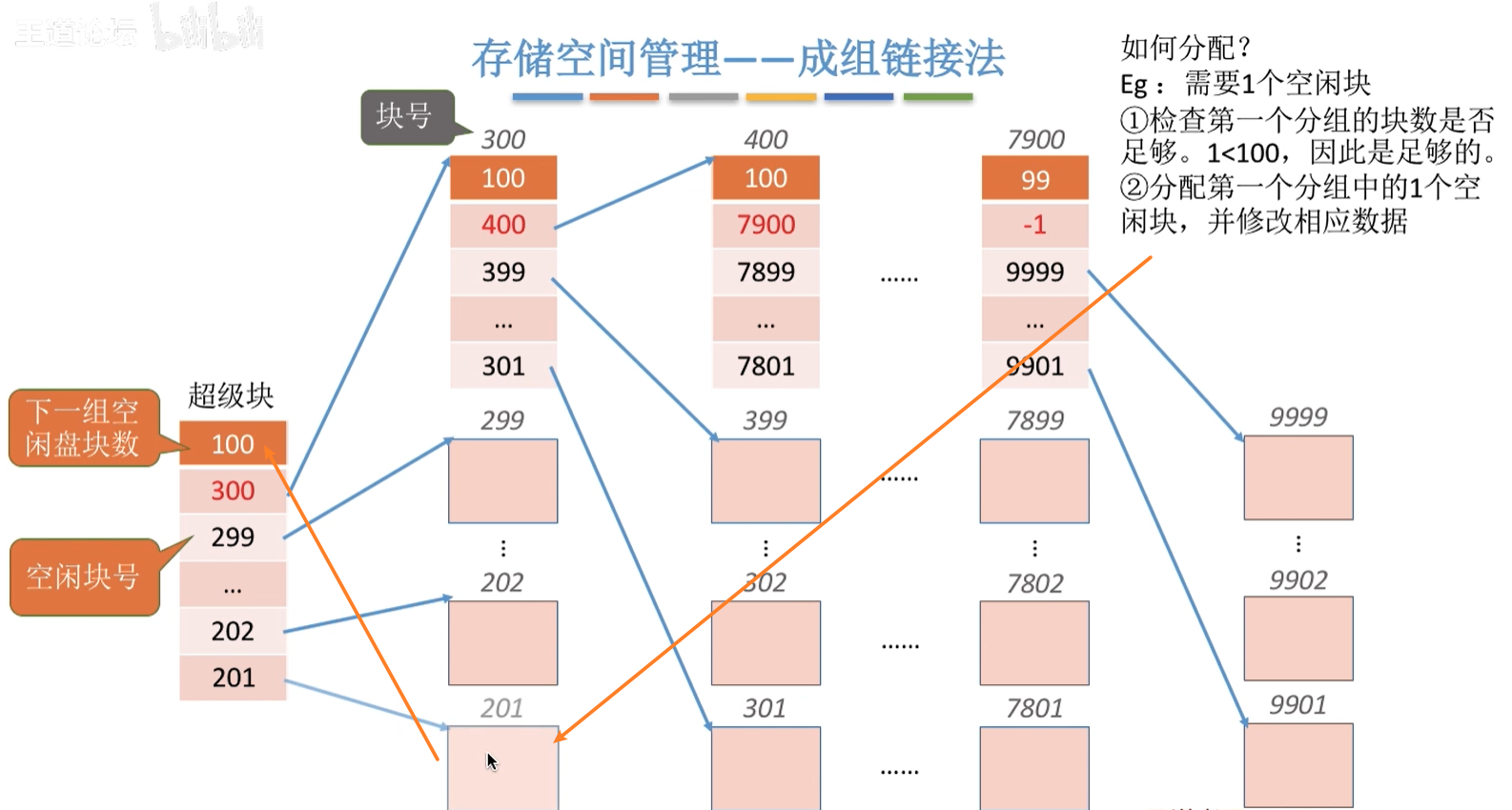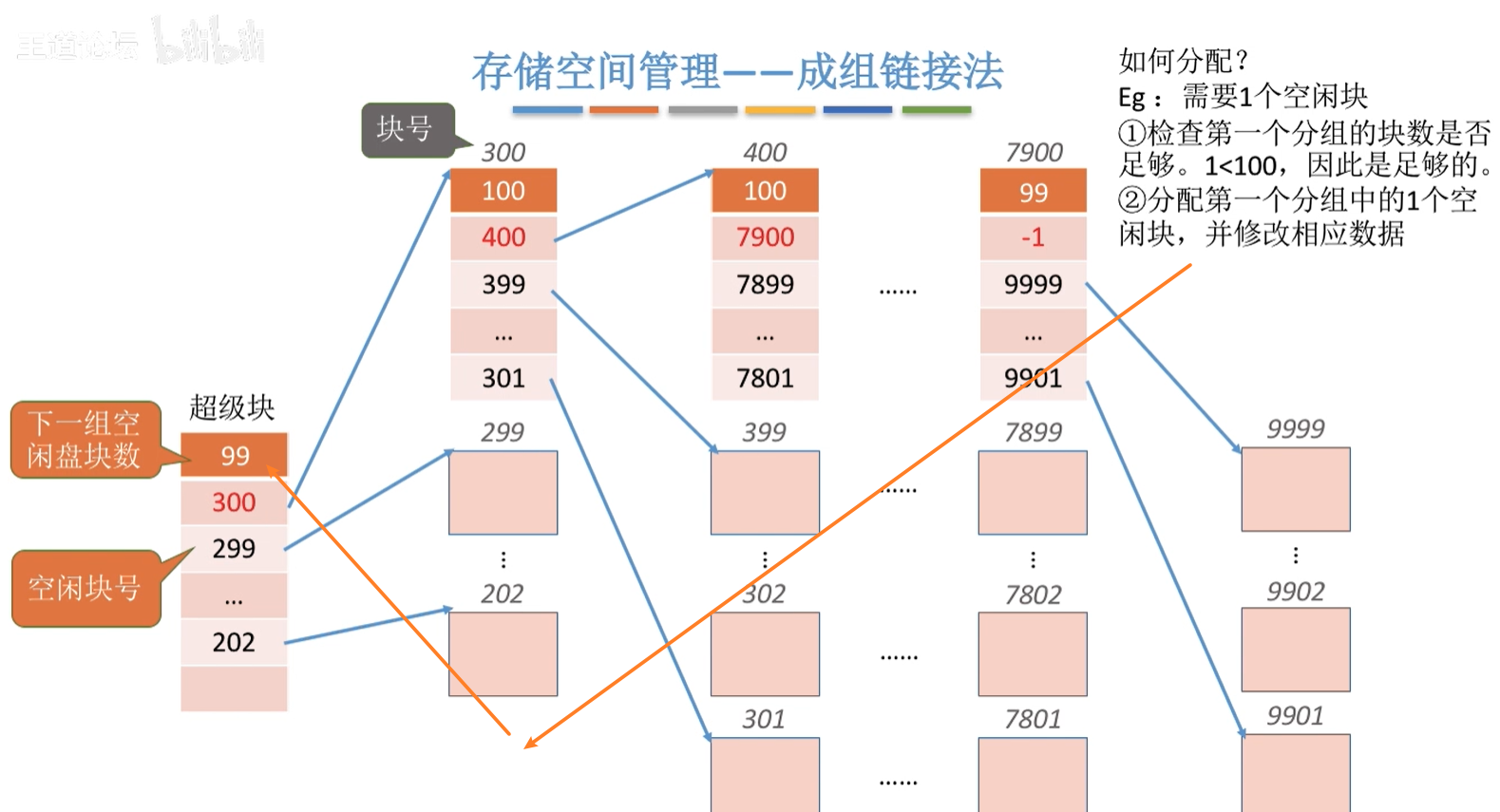需要100个空心啊磁盘块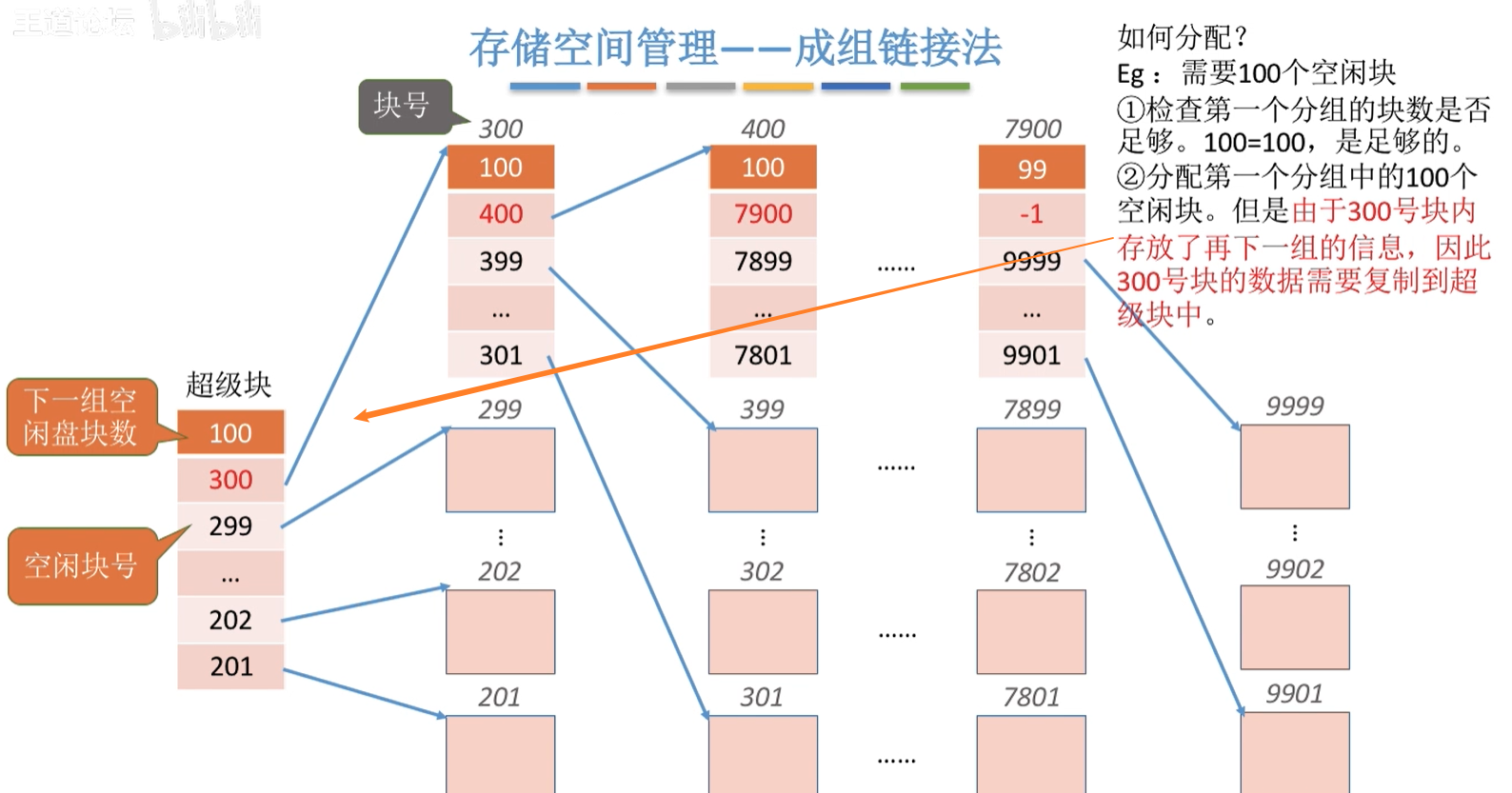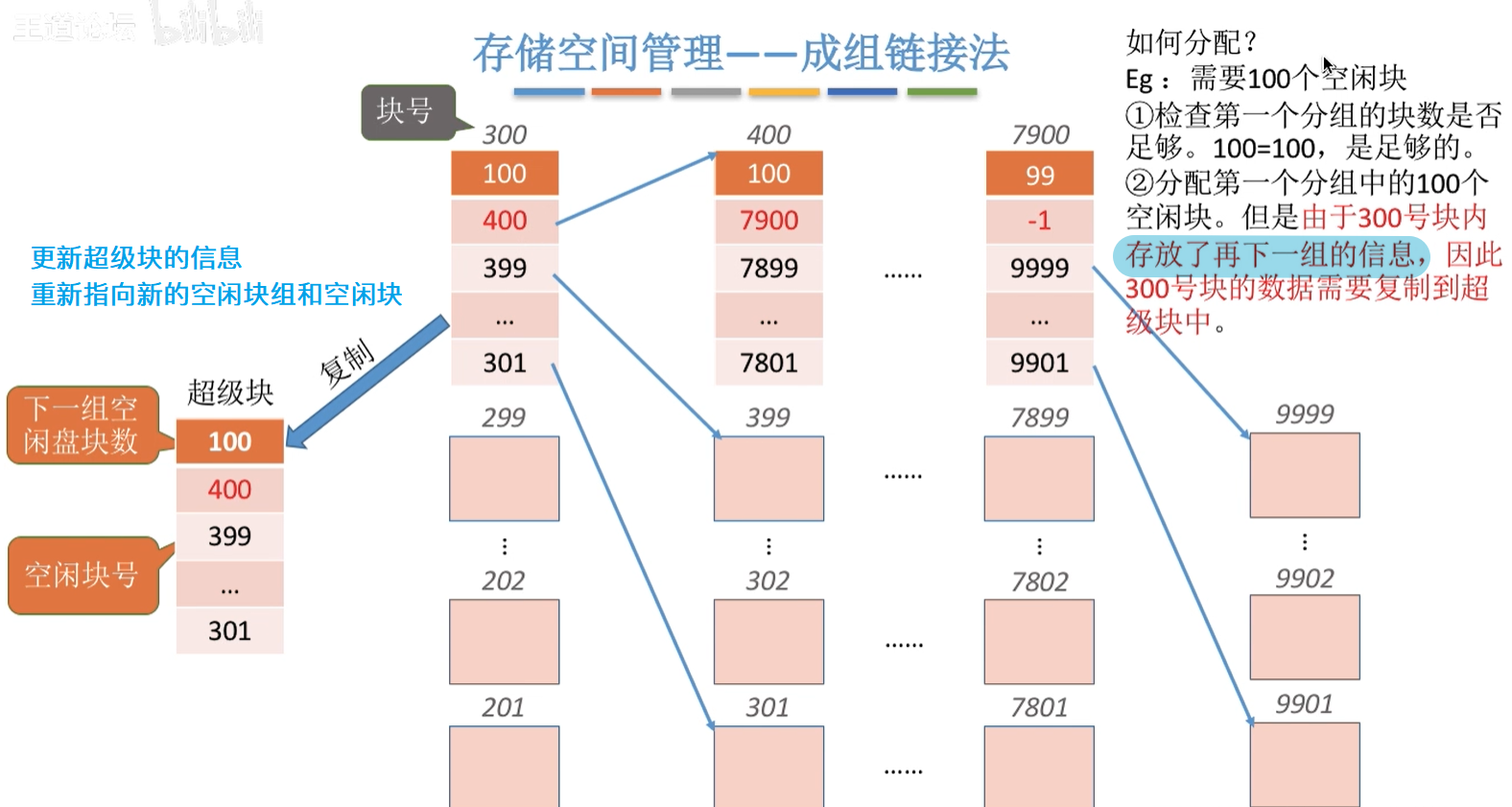如何回收？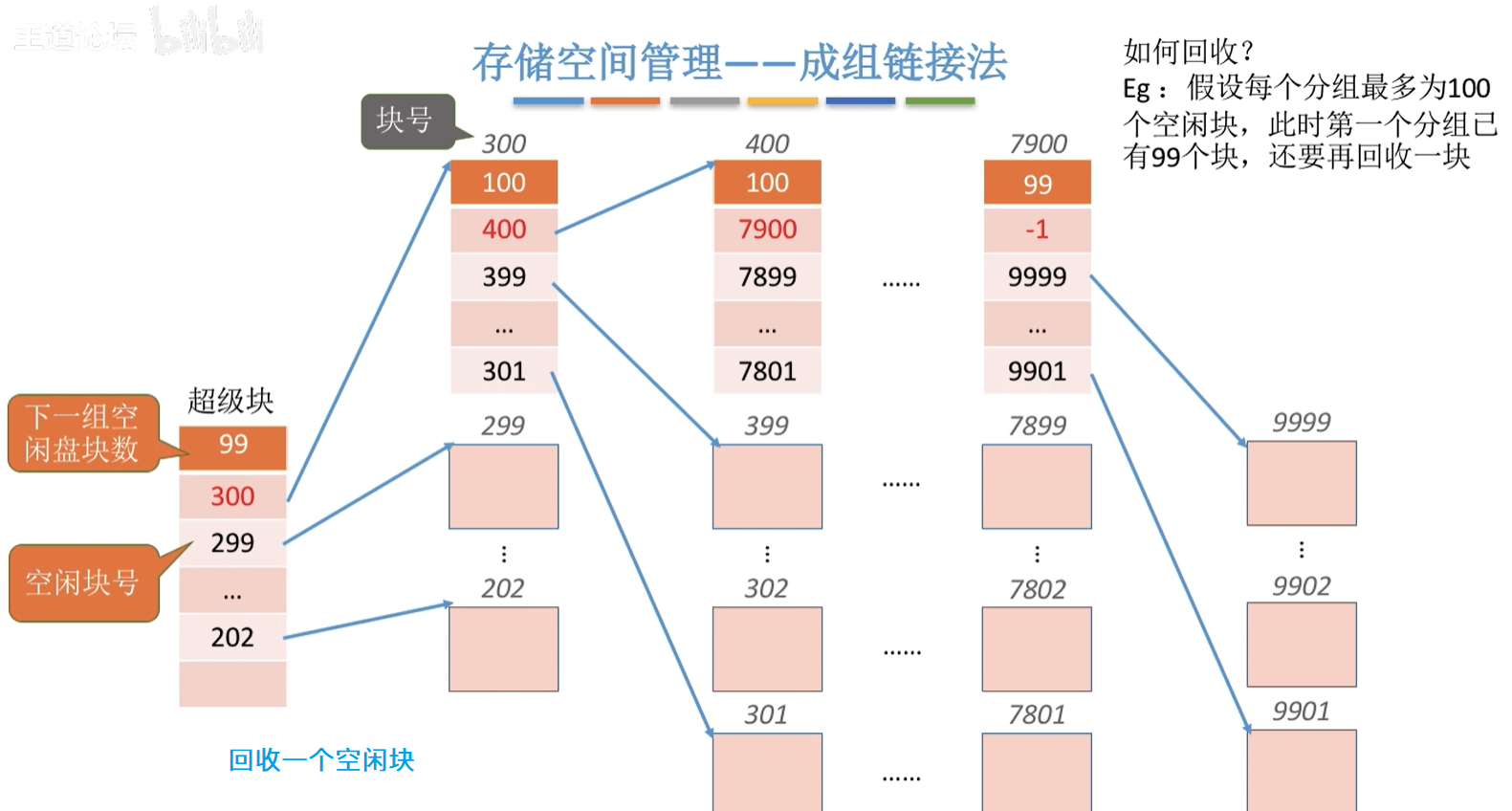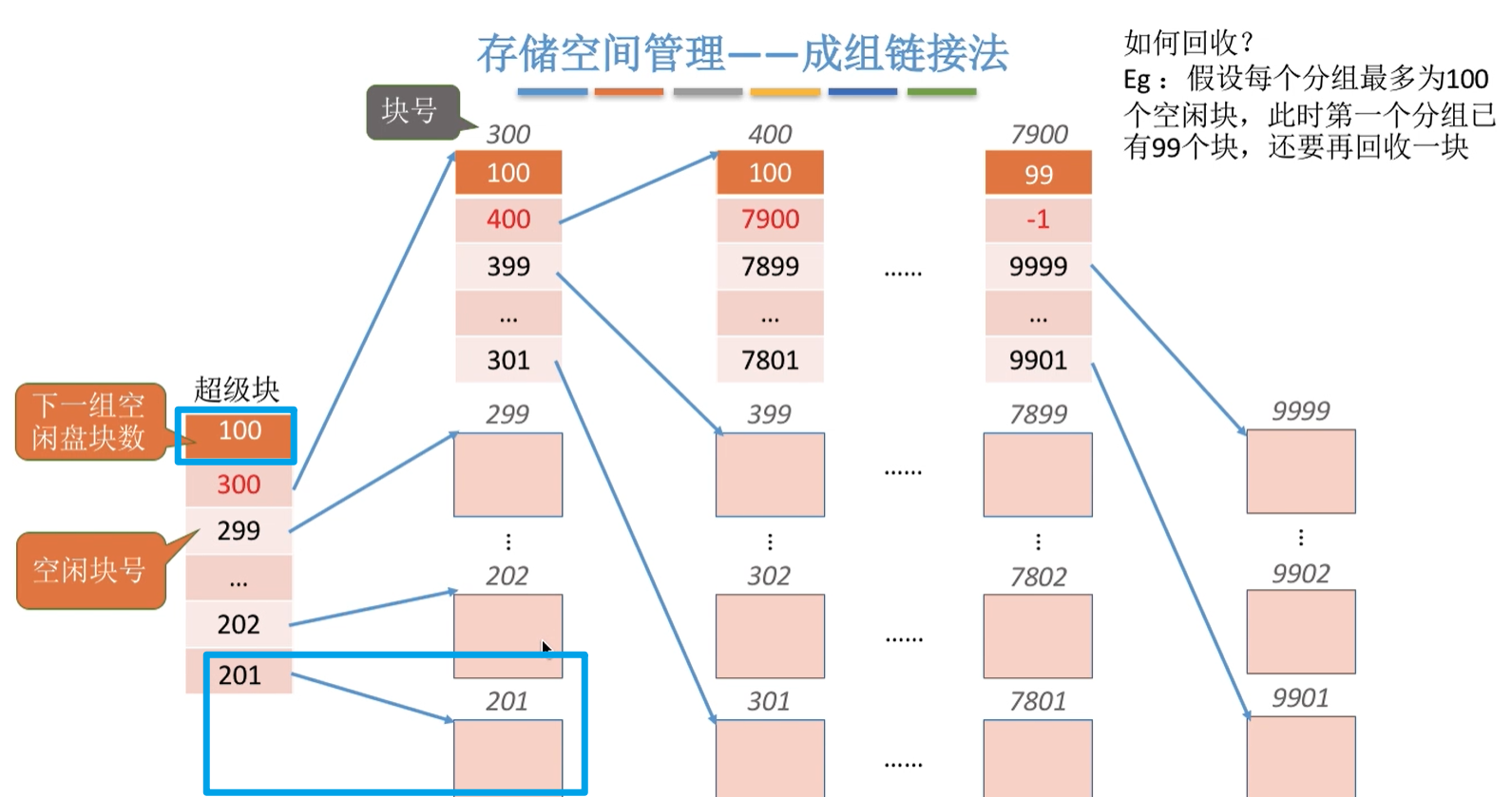第二种情况，第一组已满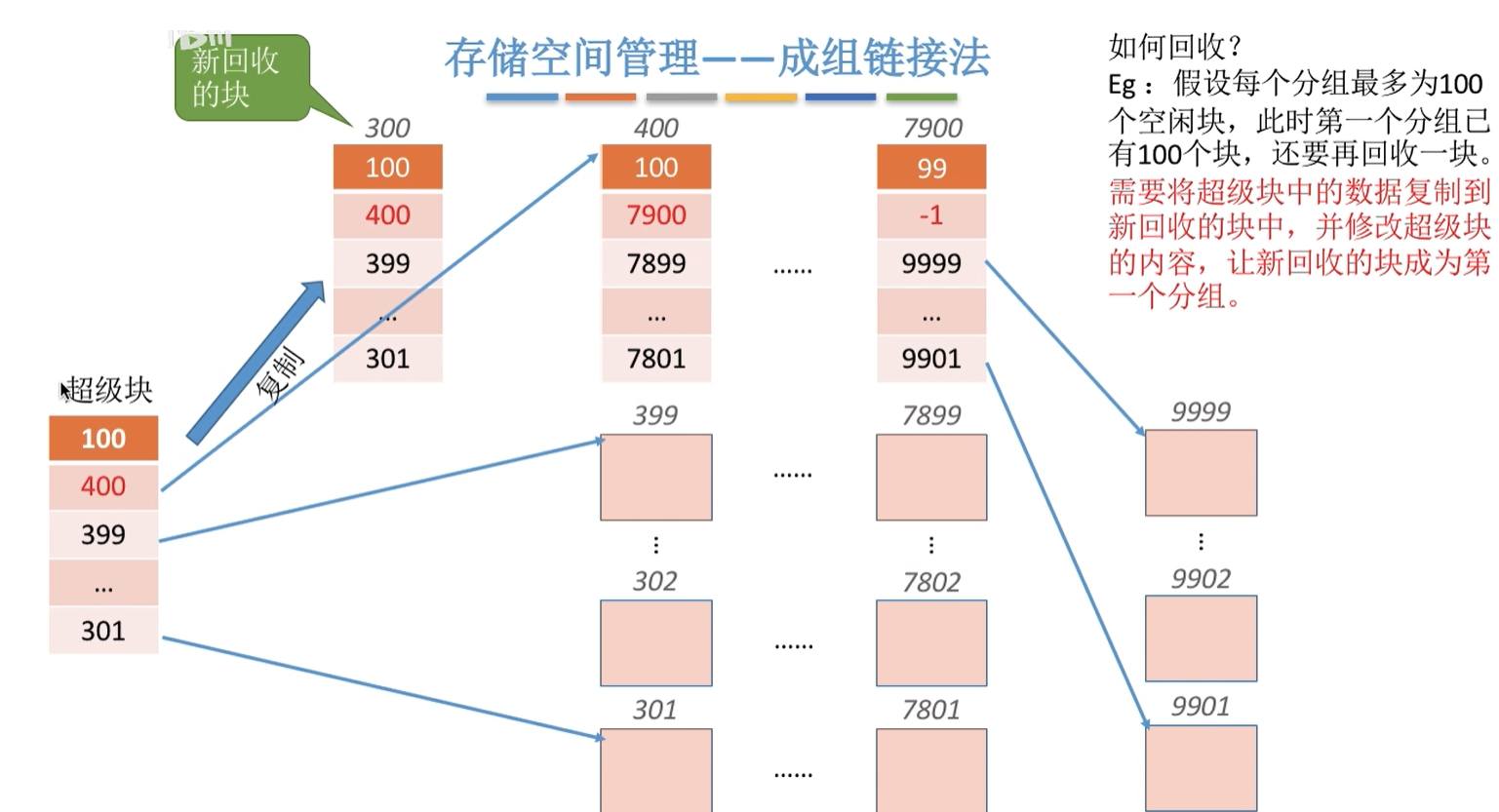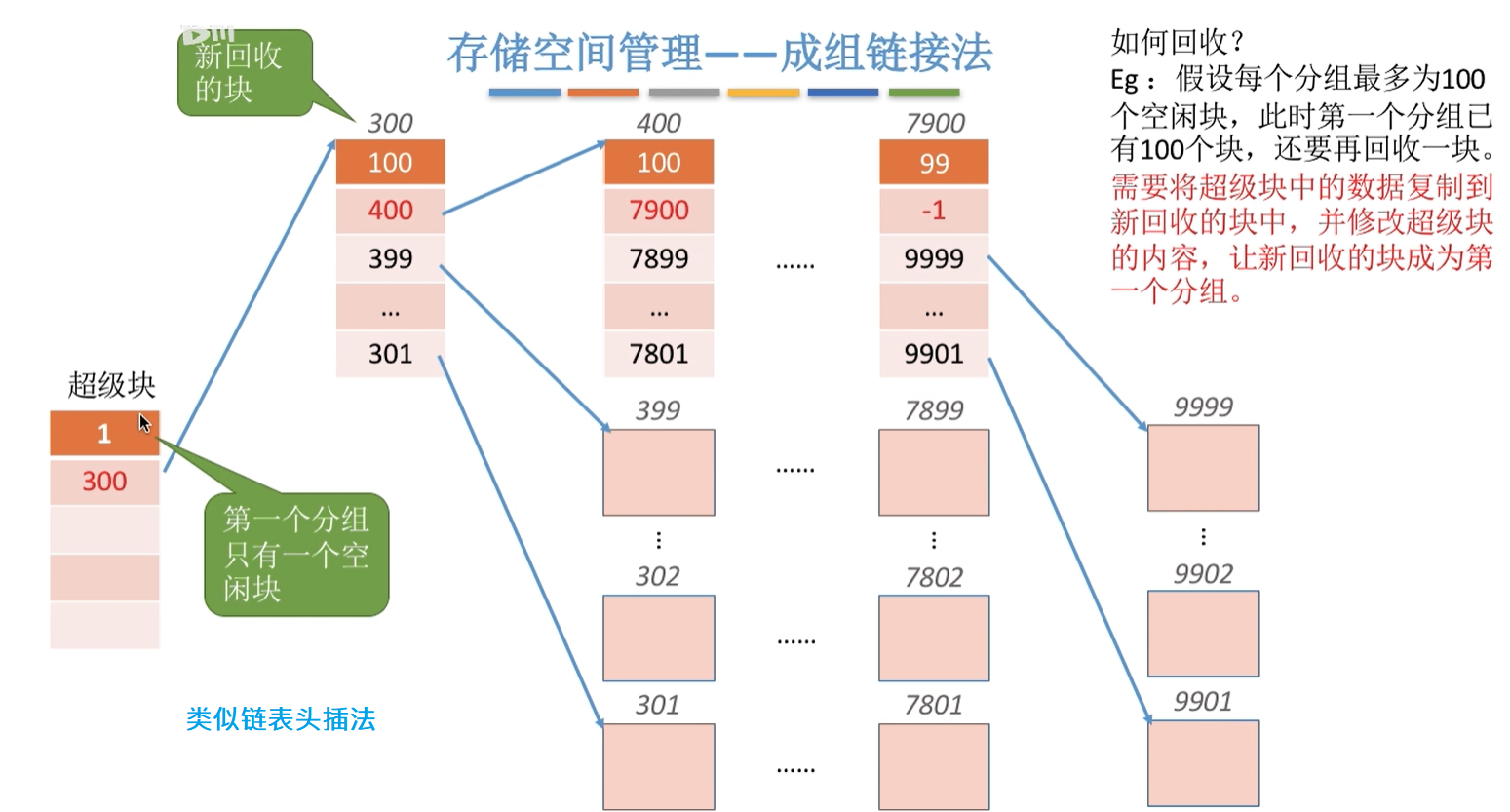参考：《王道操作系统》
展开全文文件管理 操作系统 后端 链表
• ## 实例讲解成组链接法

万次阅读 多人点赞 2017-06-22 11:20:43
成组链接法是一种用来记录磁盘空闲的方法，它使得磁盘盘的分配和回收都变得十分简单，而且没有空闲表法和空闲链表法它们的表太长的缺点，因此被引用到UNIX系统当中。 成组链接法介绍计算机上的文件是记录在...操作系统 磁盘管理
• ## 成组链接法

万次阅读 多人点赞 2016-06-18 12:25:58
成组链接法作为文件存储空间管理方法之一(主要是空闲盘区的管理)，还有其他三种管理方法分别是：空闲表法、空闲链表法和位示图法，它克服了空闲链表法表太长的缺点，但是保持了其优点，即分配和回收一个盘比较简单...unix 磁盘 管理 操作系统
• 成组链接法介绍 在UNIX系统中，将空闲块分成若干组，每100个空闲块为一组，每组的第一空闲块登记了下一组空闲块的物理盘块号和空闲块总数。 在UNIX系统中，将空闲块分成若干组，每100个空闲块为一组，每组的第一...unix 操作系统
• 模拟unix系统成组链接法，实现磁盘存储空间的管理，假定共有8可供使用，每3为一组。
• 4、文件存储空间管理思维导图文件的初始化和划分文件存储空间管理方法1、存储空间管理——空闲表法2、存储空间管理——空闲链表法3、存储空间管理——位示图法4、存储空间管理——成组链接法 思维导图 文件的初始化...
• 成组链接法是Unix系统中常见的管理空闲盘区的方法。   在UNIX系统中，将空闲块分成若干组，每100个空闲块为一组，每组的第一空闲块登记了下一组空闲块的物理盘块号和空闲块总数。如果一个组的第二个空闲块号等于0...操作系统 unix 文件存储管理
• 成组链接法是结合了空闲表和空闲链表法的，UNIX系统采用的就是成组链接法成组链接法中保存的是当前可用的存储盘的地址，具体的我们以一个简单的例子来阐述他的结构和分配回收原理。 前提假设 假设一个空闲的盘...操作系统
• 下面将介绍比较实用又有点复杂的成组链接法，看它是如何把磁盘中所有的空闲都记录起来，又不耗费太多的内存空间。  请看下图：  下面的文字来自汤氏的操作系统教材： 1、空闲的组织 （1）空闲号栈...j...